# MSBSHSE Solutions For SSC (Class 10) Maths Part 2 Chapter 7 - Mensuration

MSBSHSE Solutions For SSC (Class 10) Maths Part 2 Chapter 7 – Mensuration are given here with step by step explanations and clear diagrams. All solutions from MSBSHSE Solutions For SSC (Class 10) Maths Part 2 Chapter 7 are provided here for free. BYJU’S provides you accurate solutions, which are prepared by our subject matter experts. These solutions help the students clear understanding of each and every step. This chapter of Maharashtra State Board Class 10 Solutions deals with surface area and volume of three dimensional figures such as cone, cylinder, sphere, hemisphere and frustum of cone. These solutions help students to build a strong base of concepts .

## Download the PDF of Maharashtra Solutions For SSC Maths Part 2 Chapter 7 Mensuration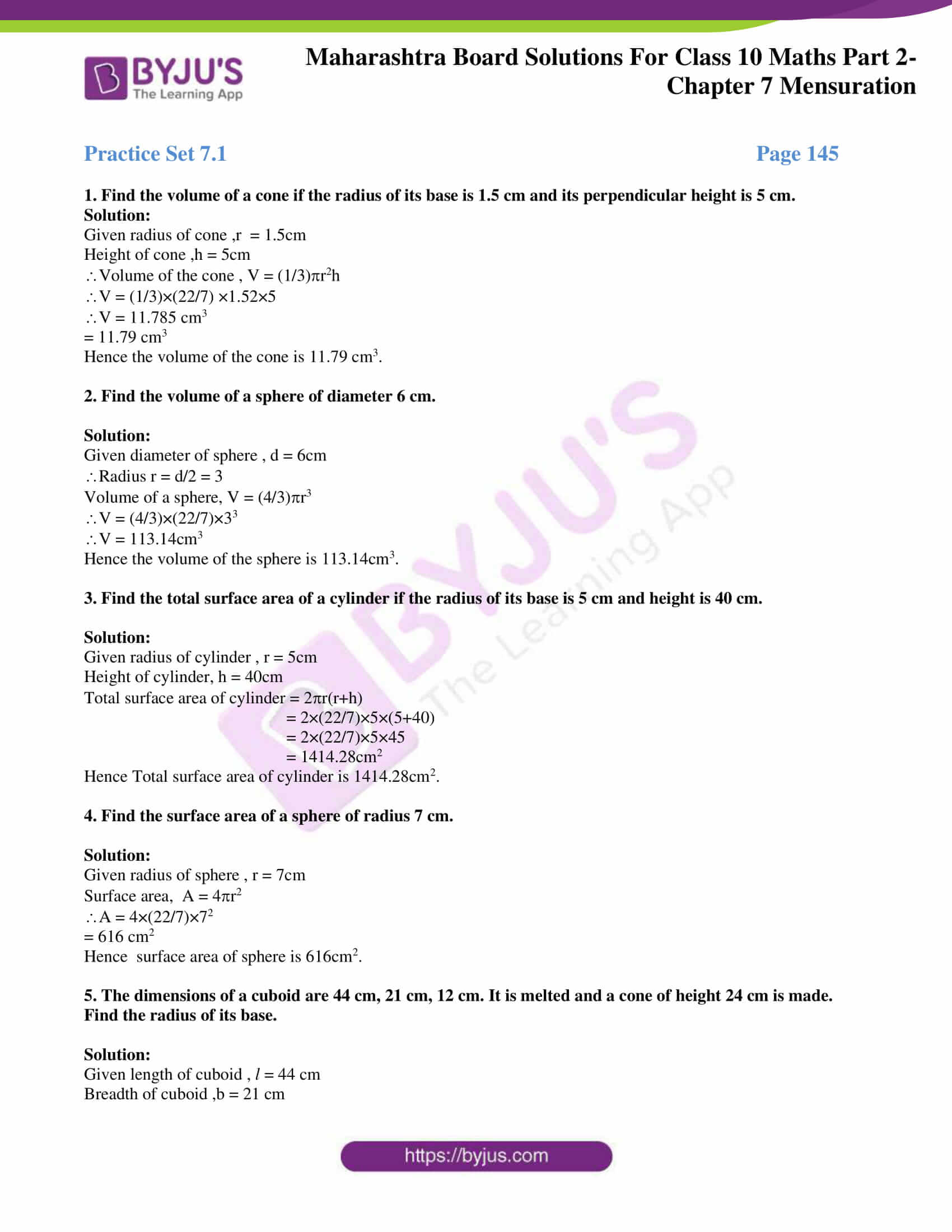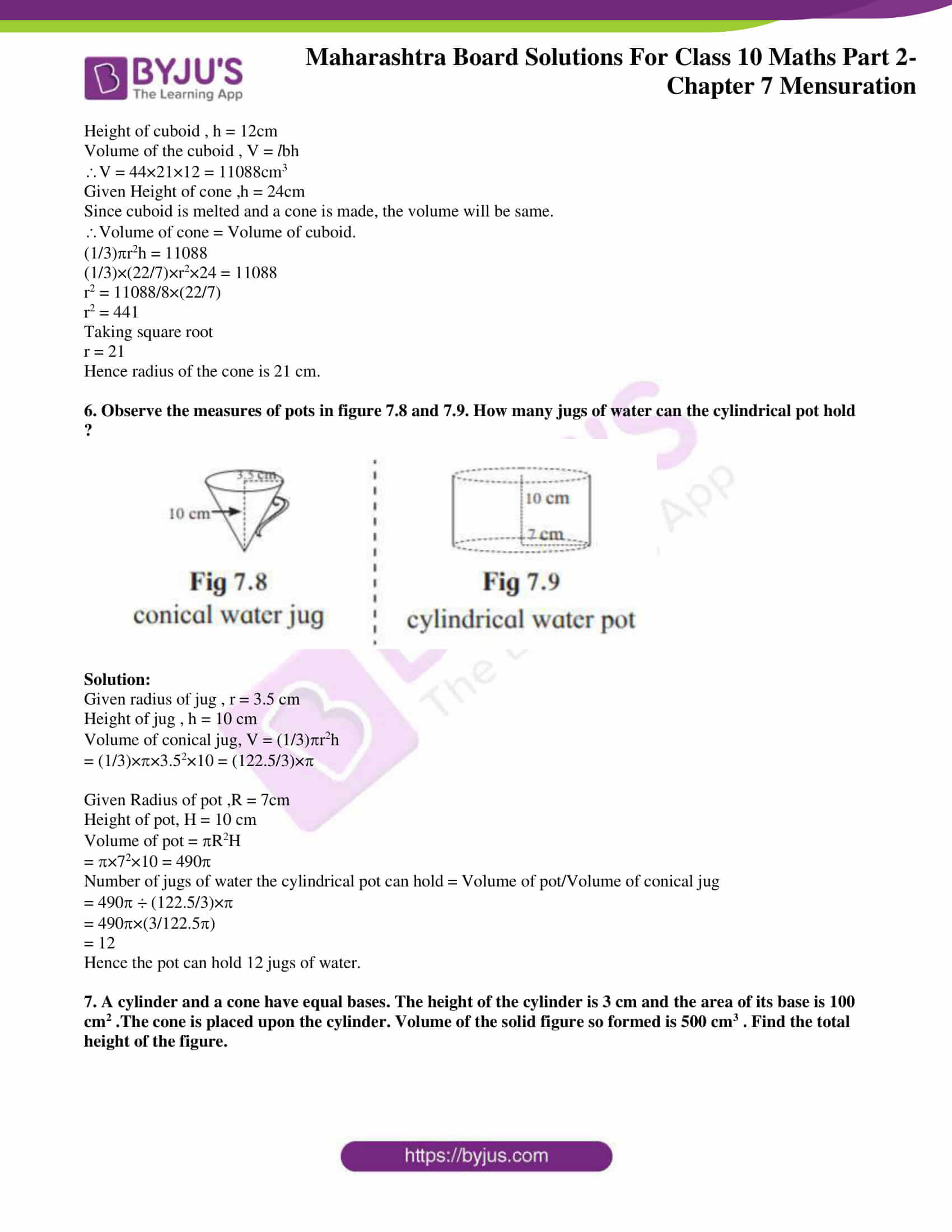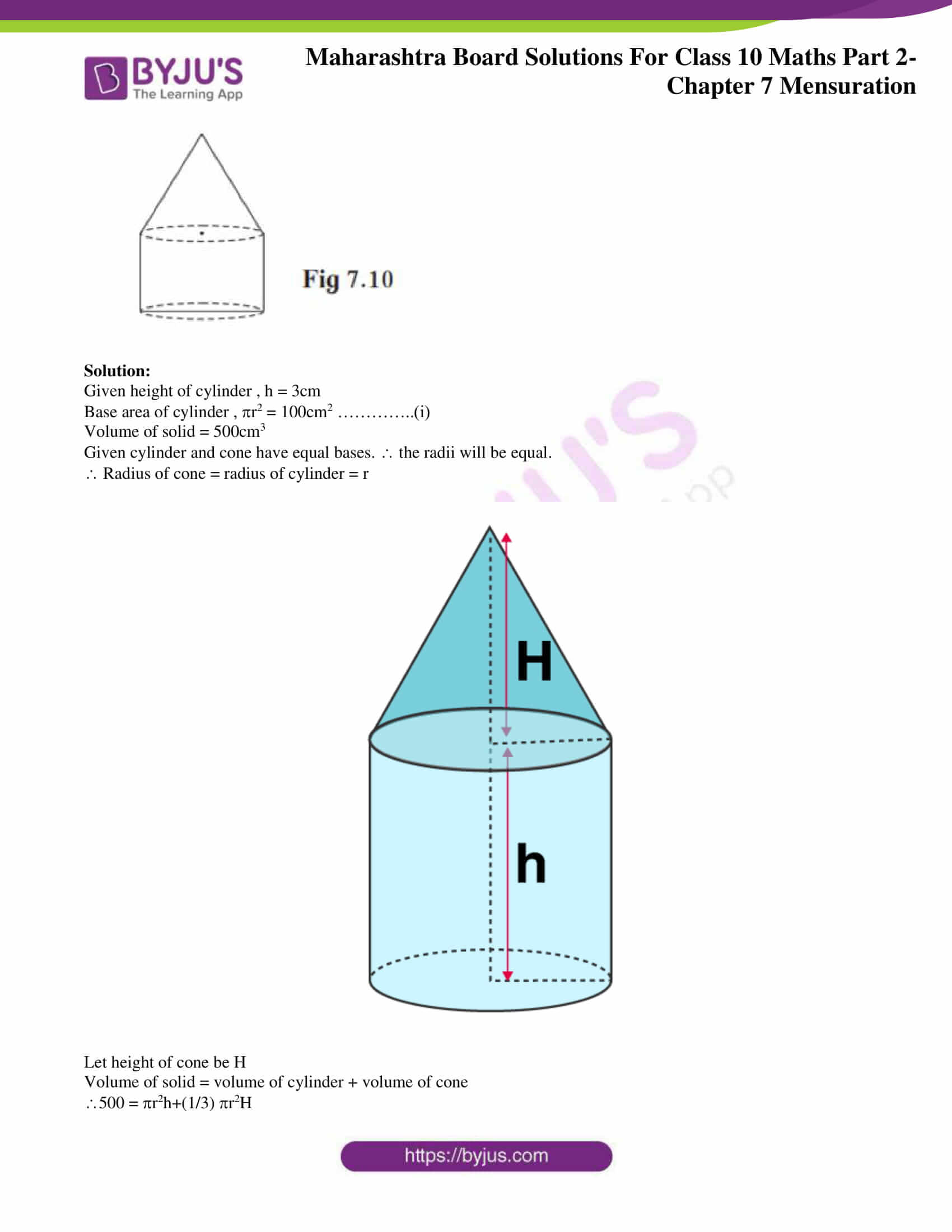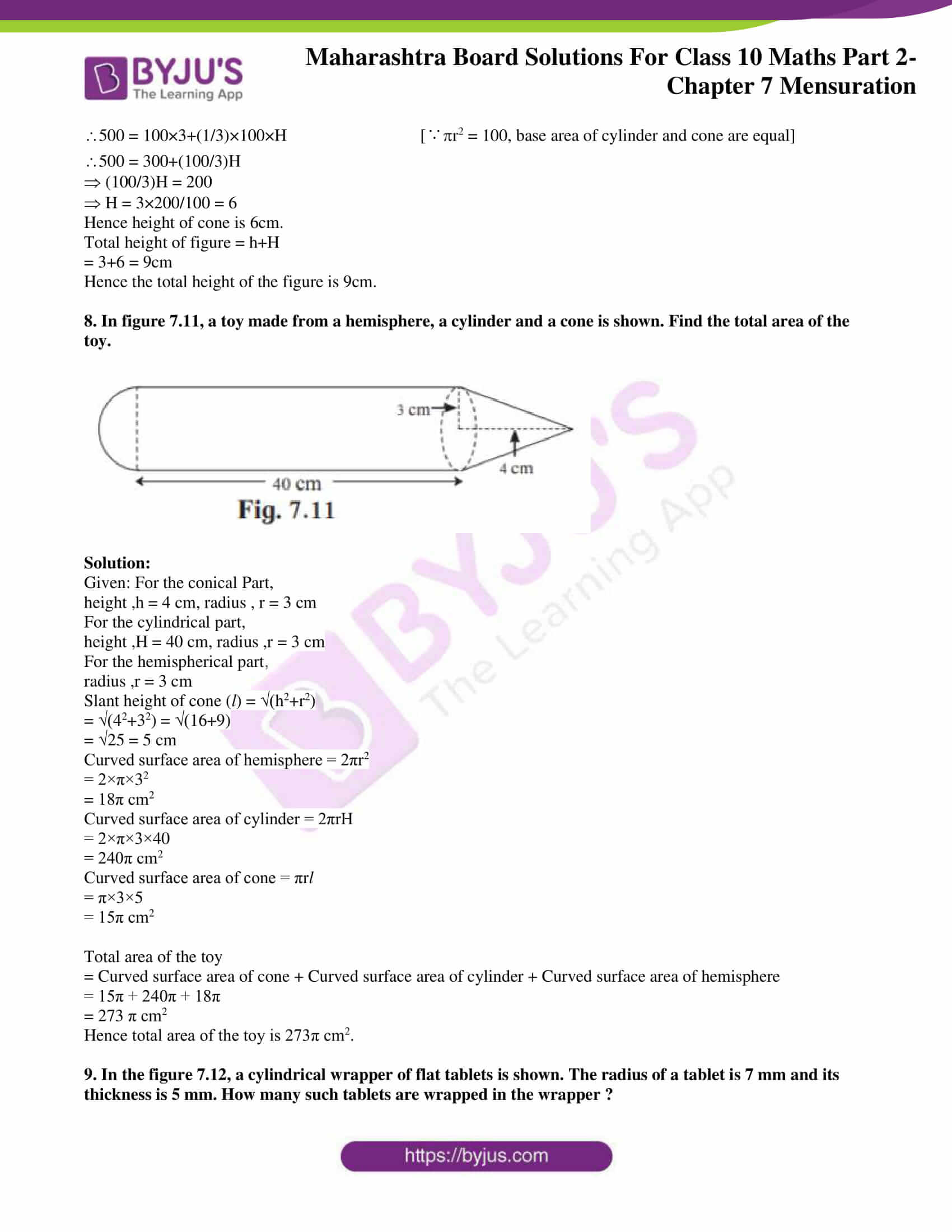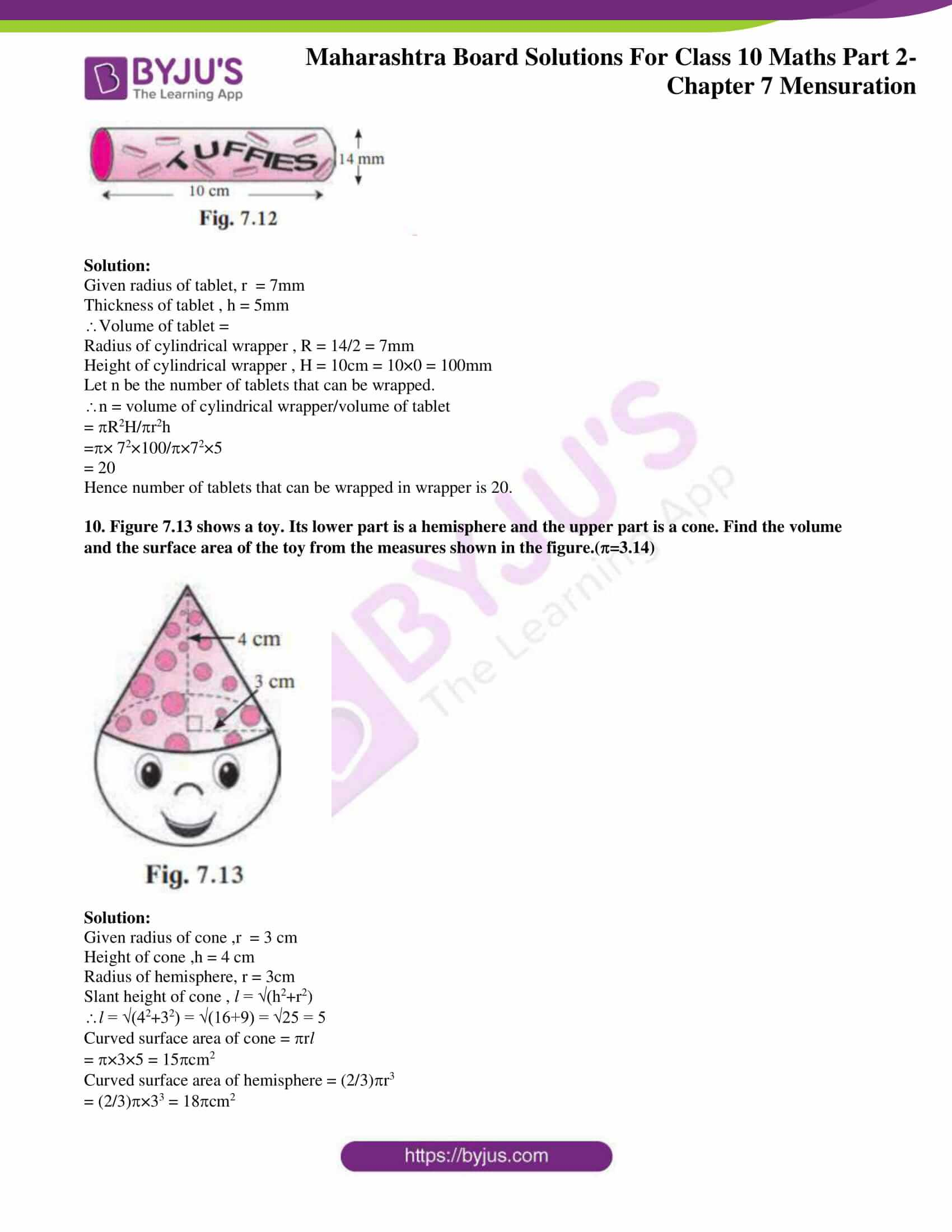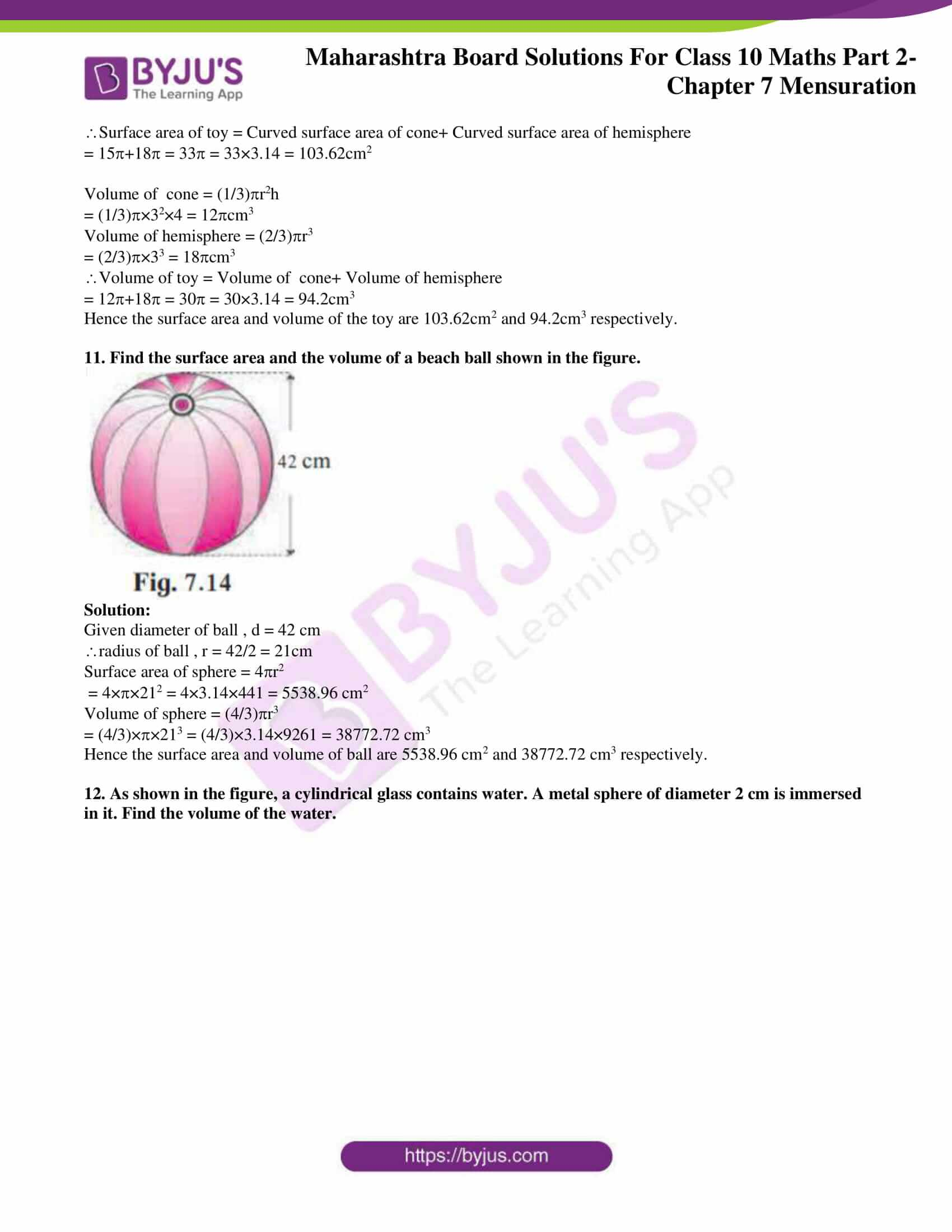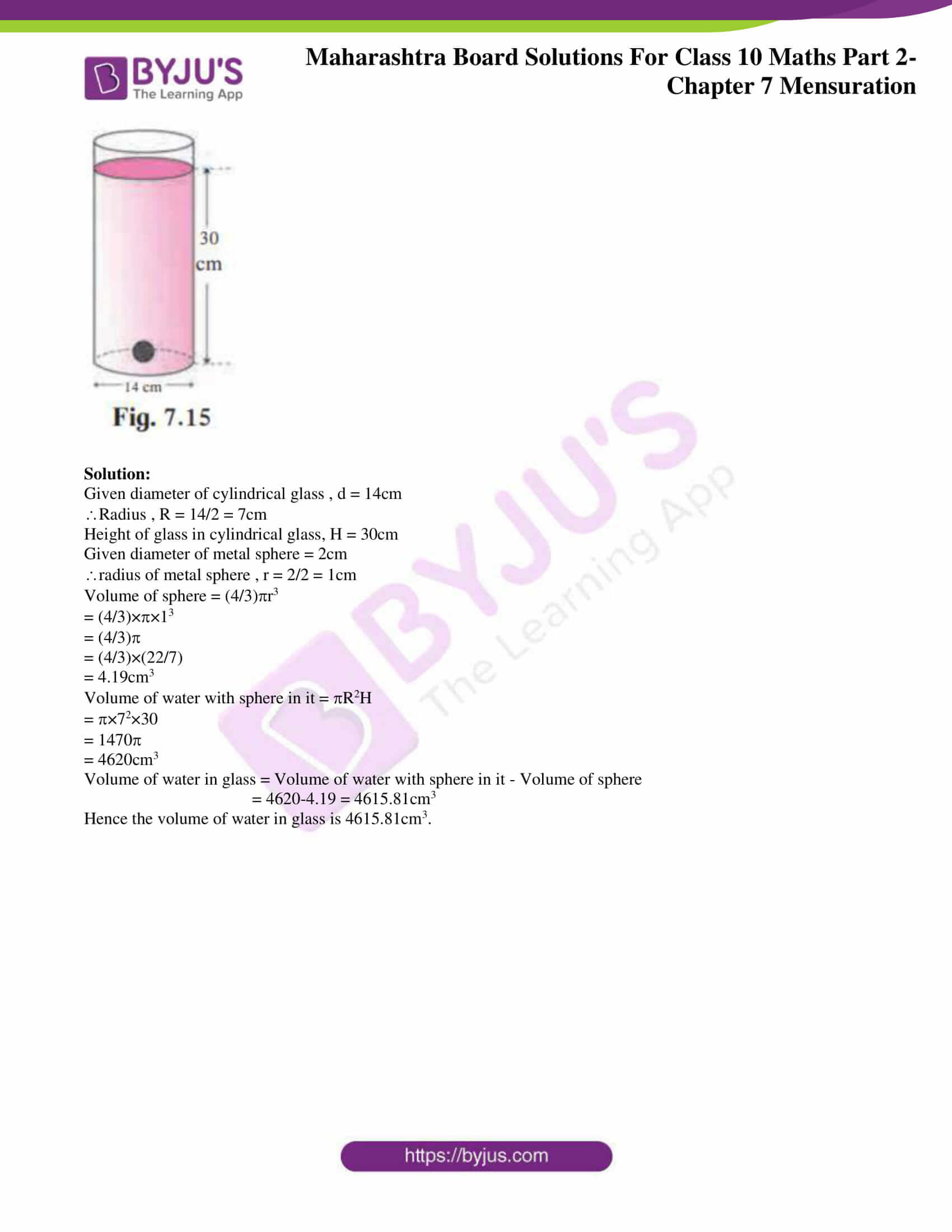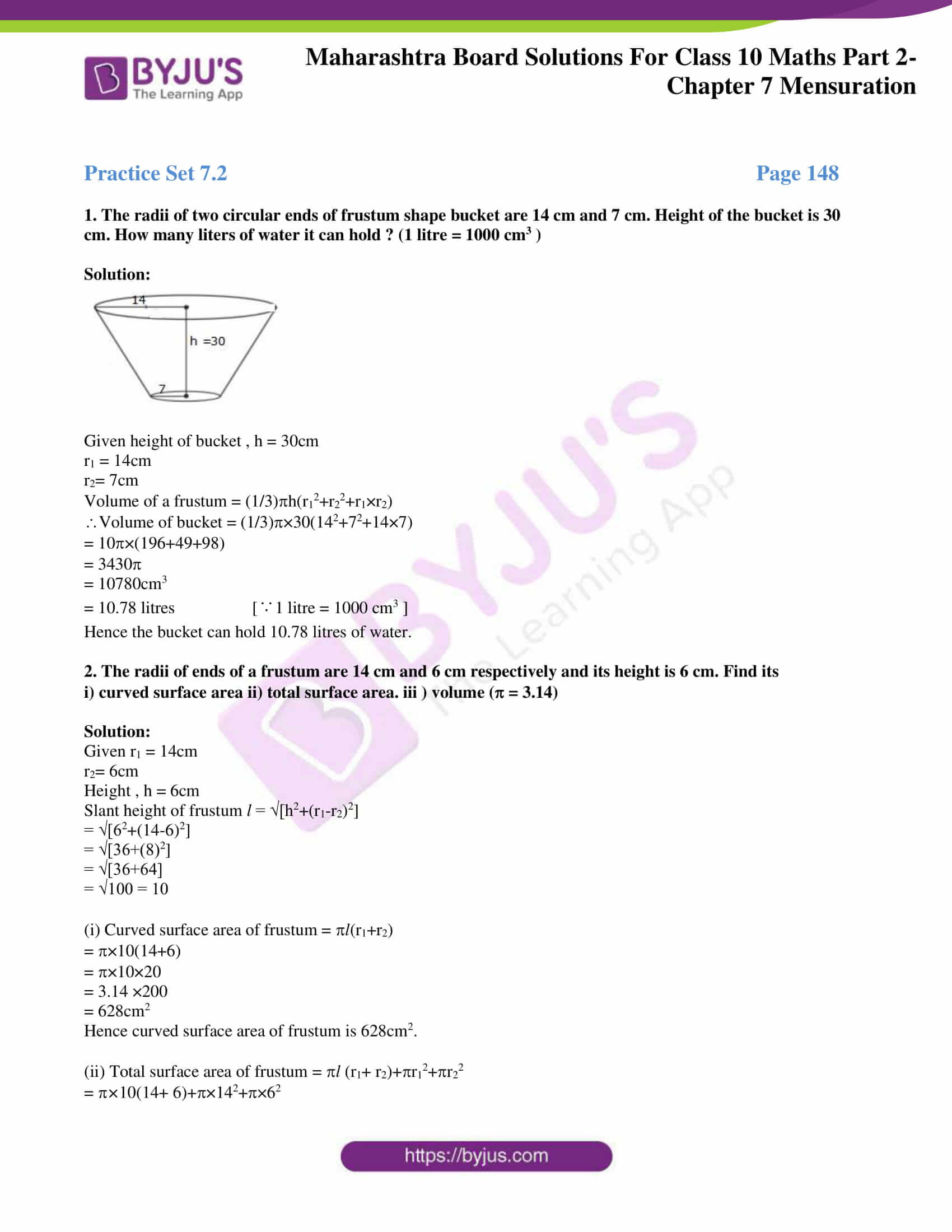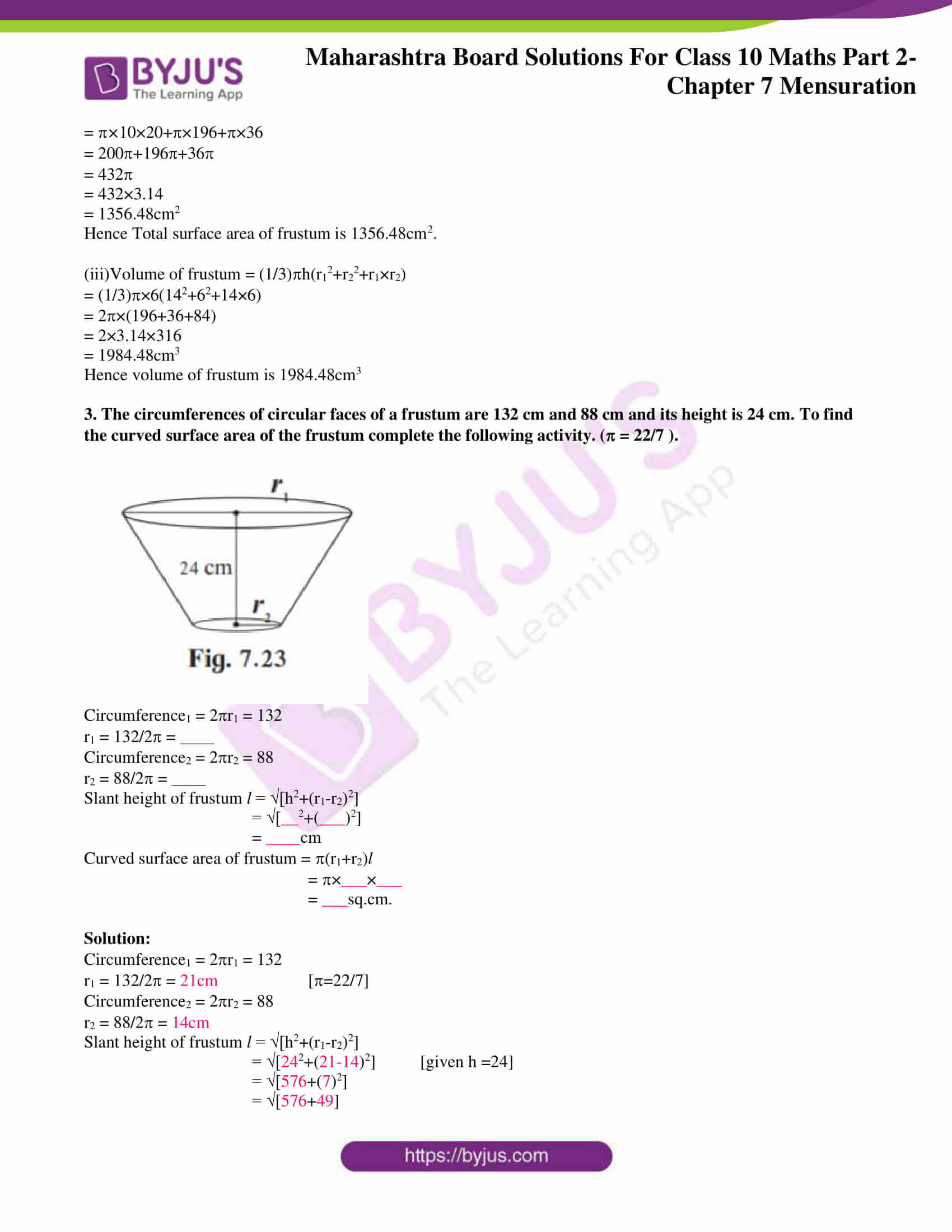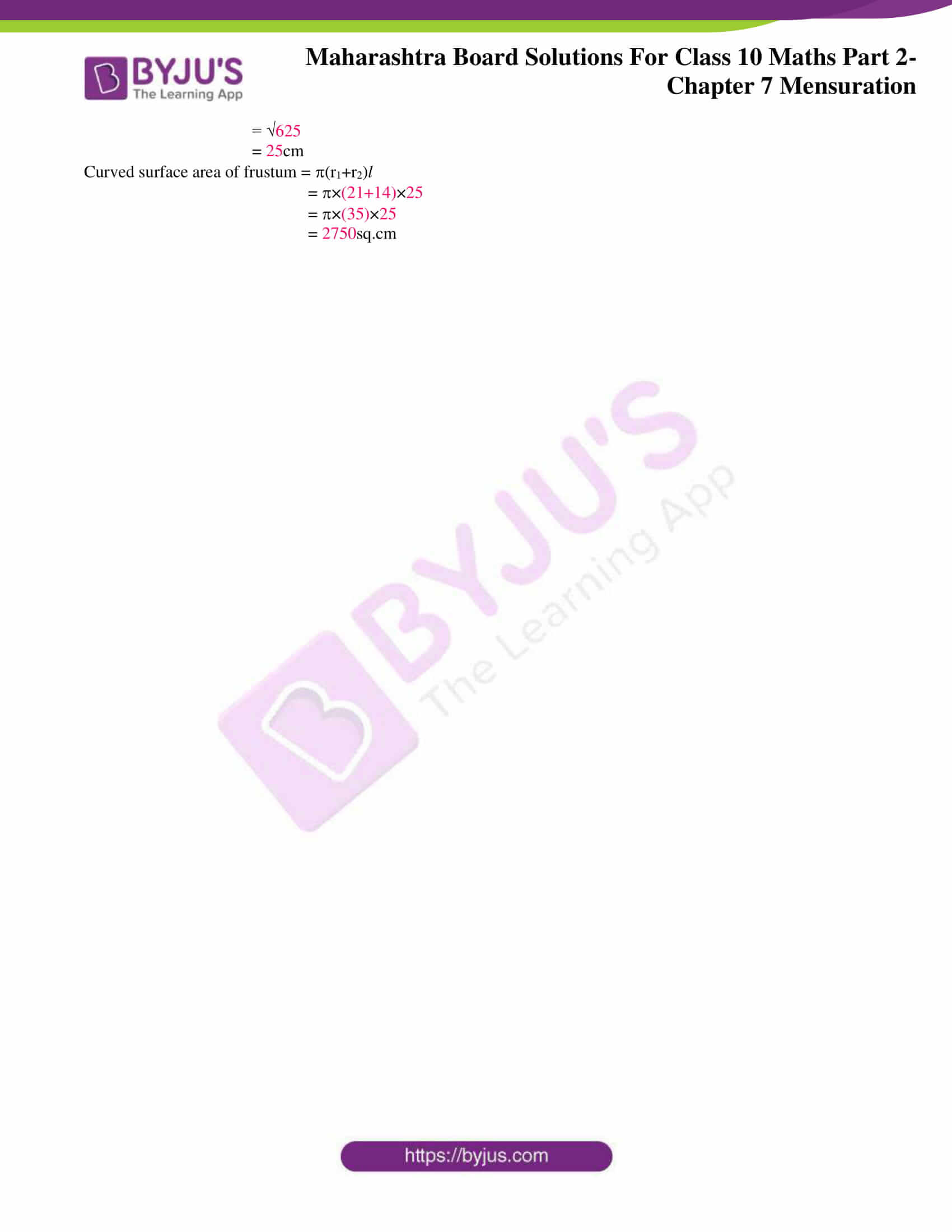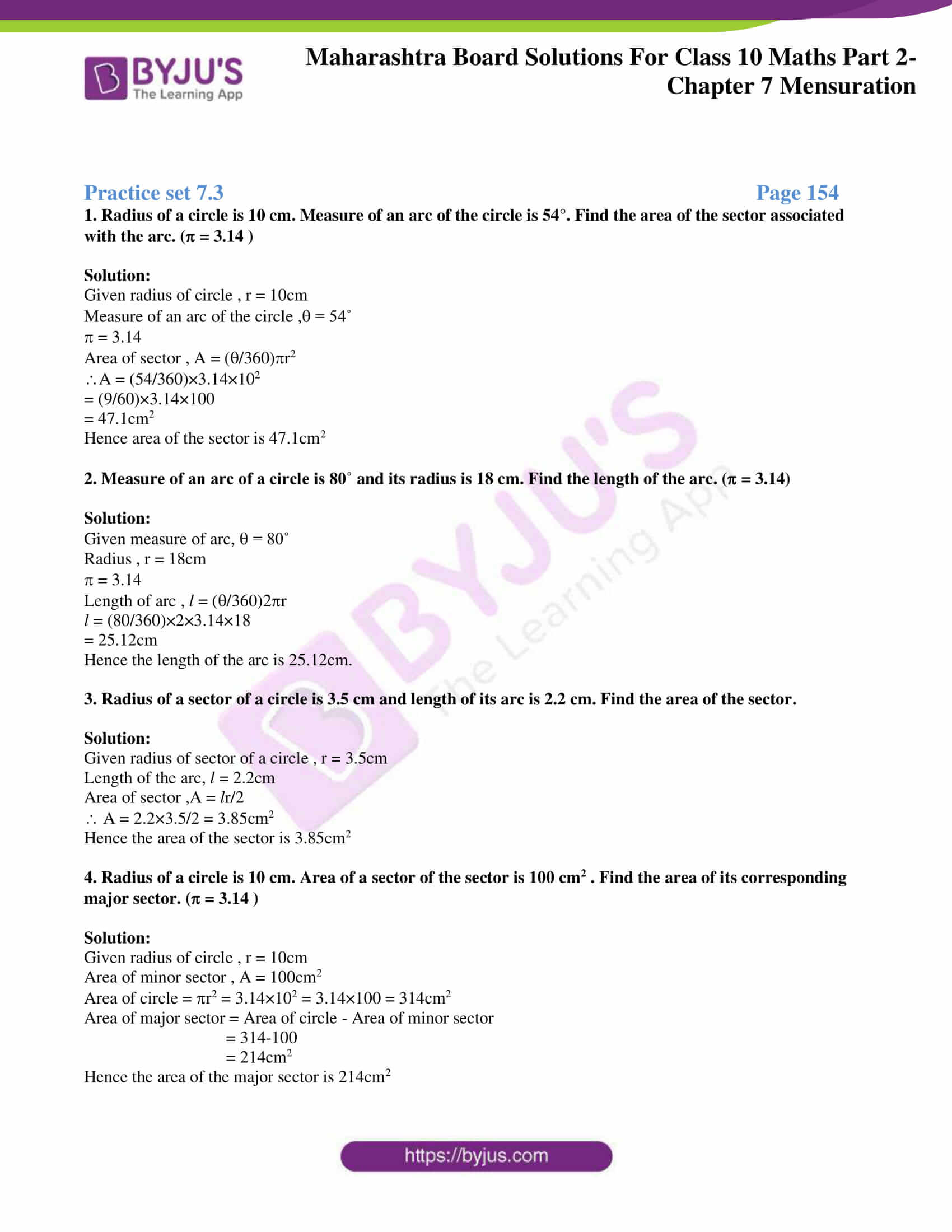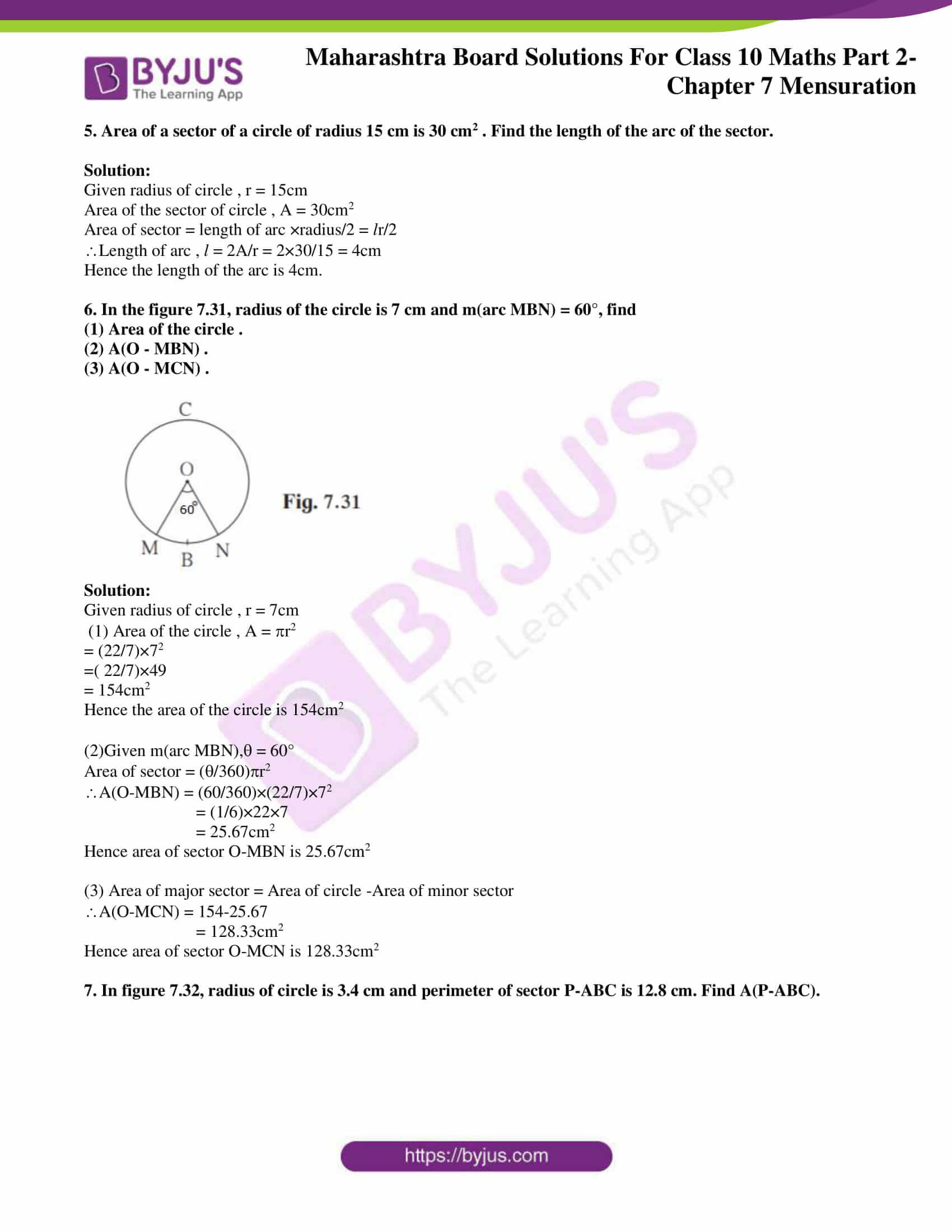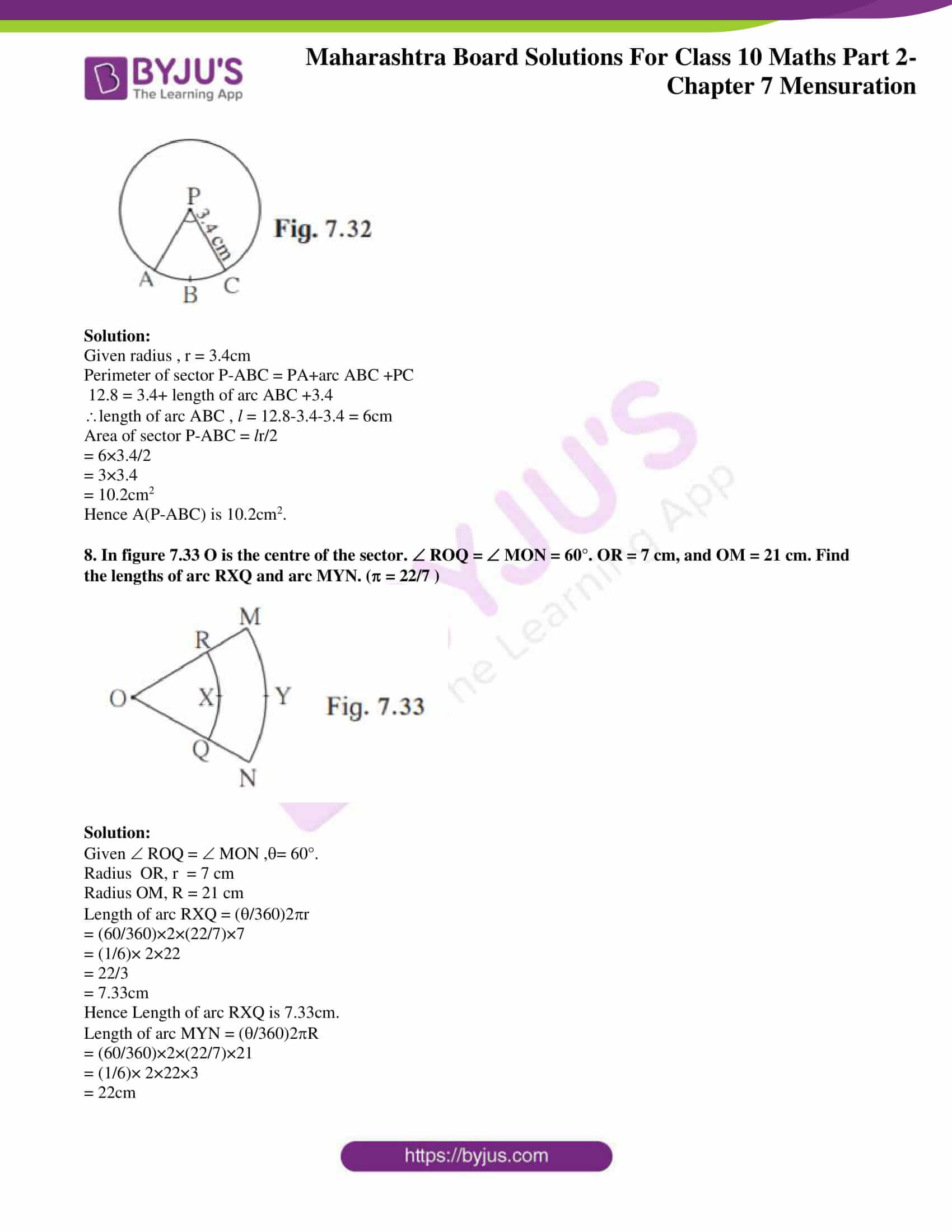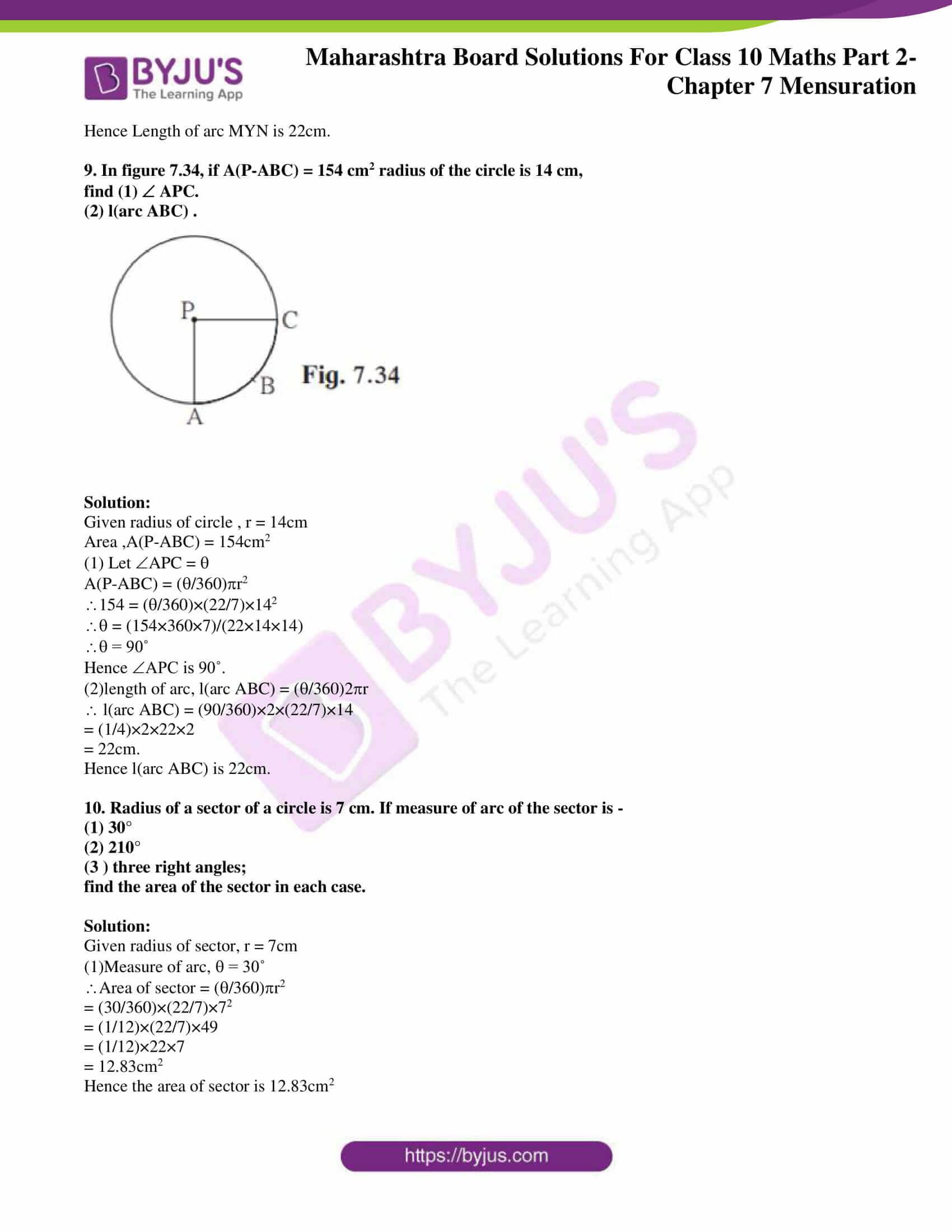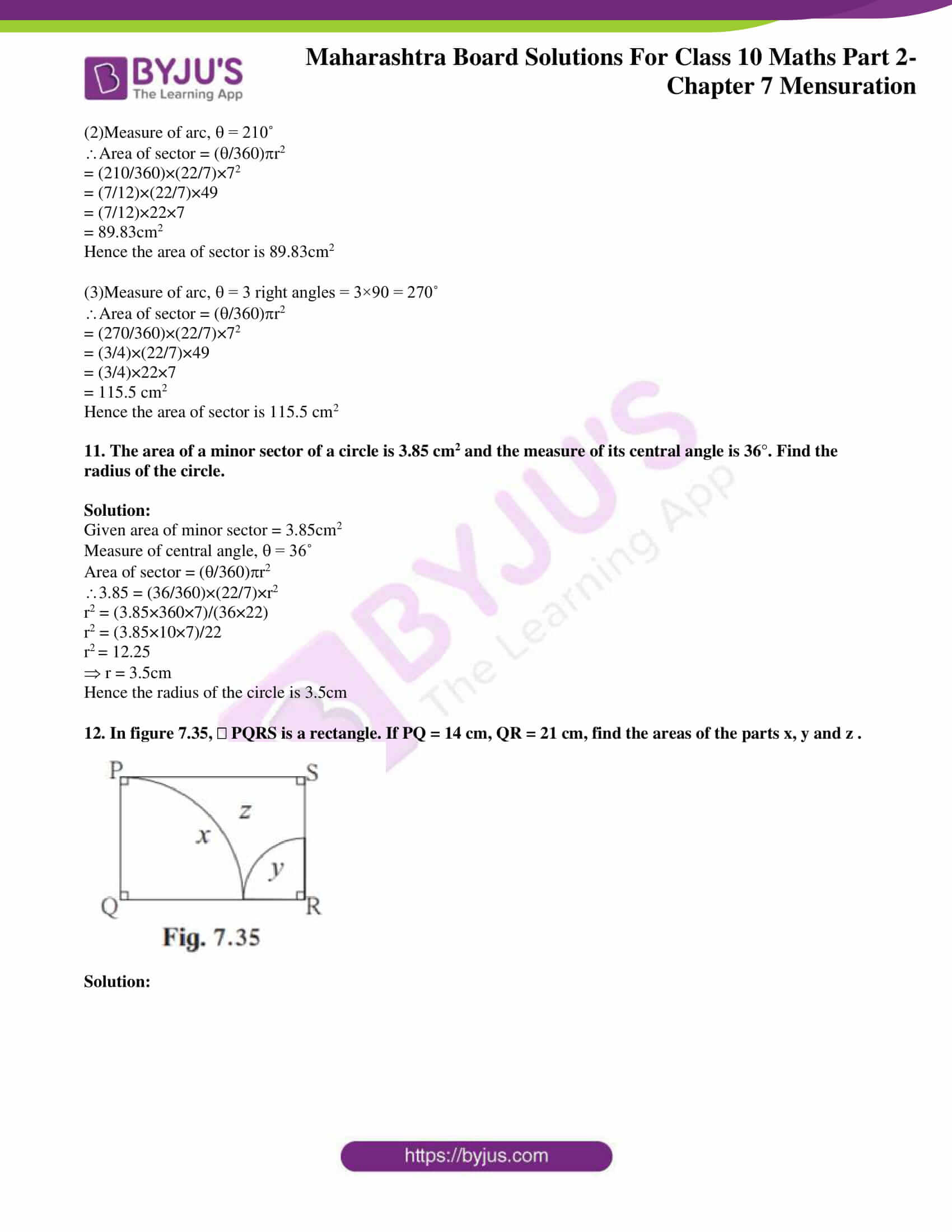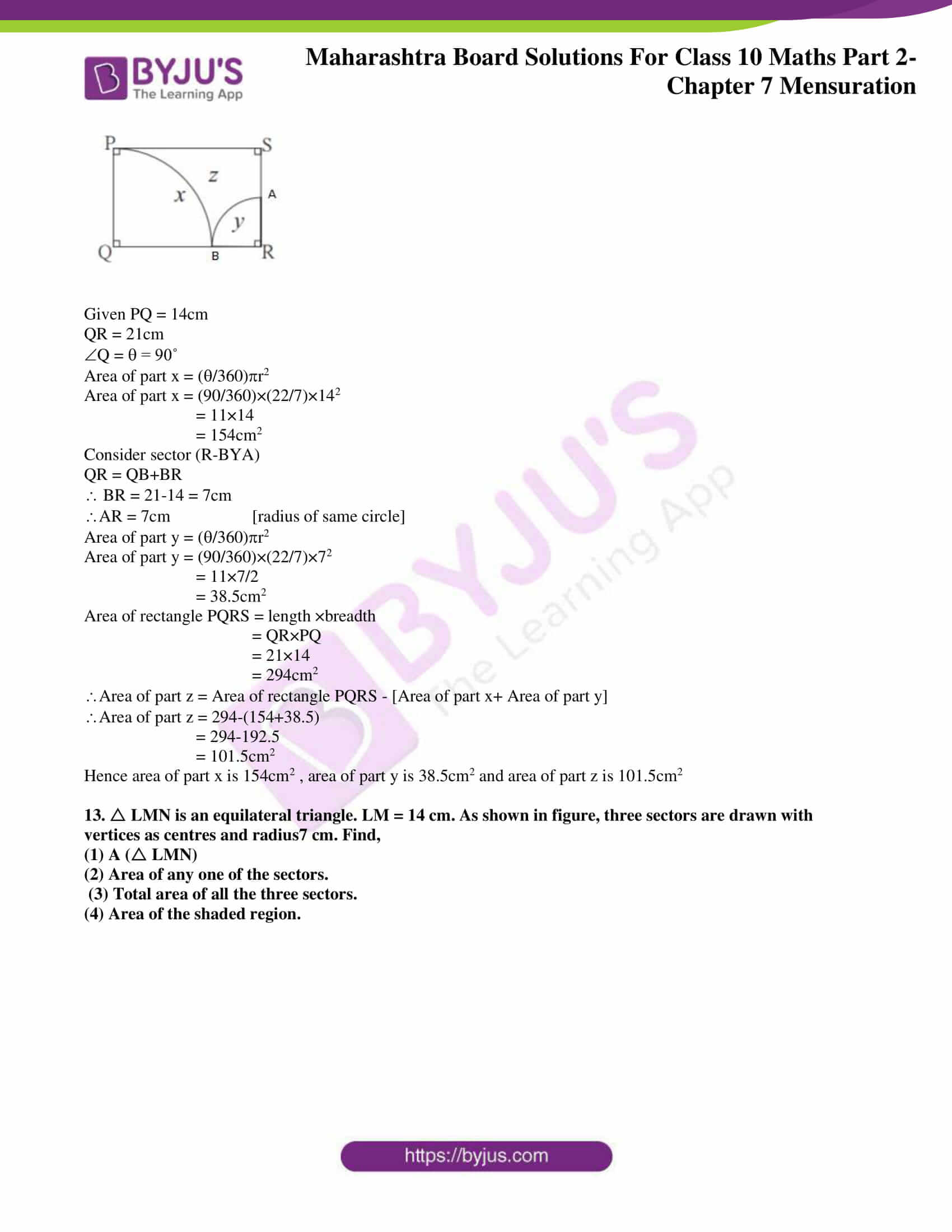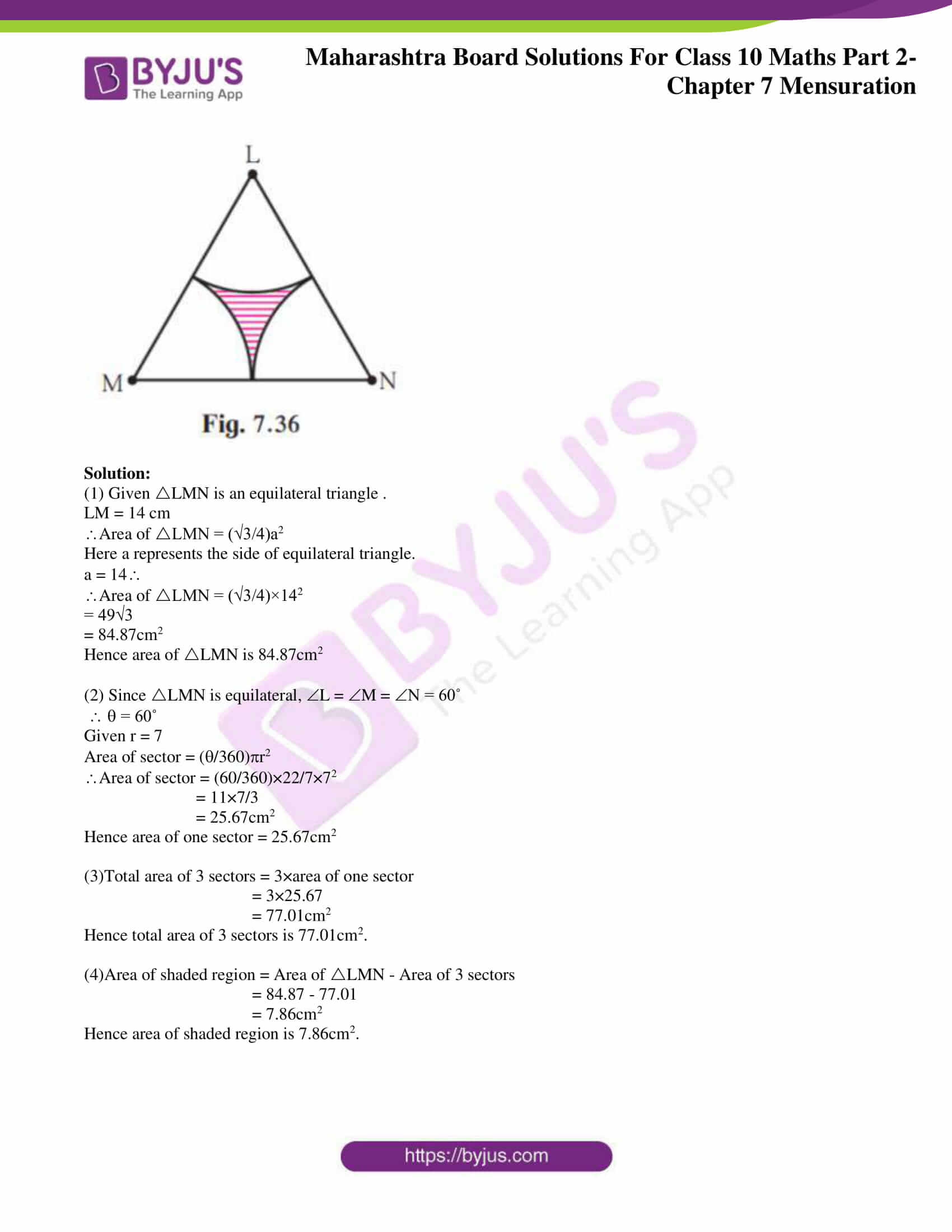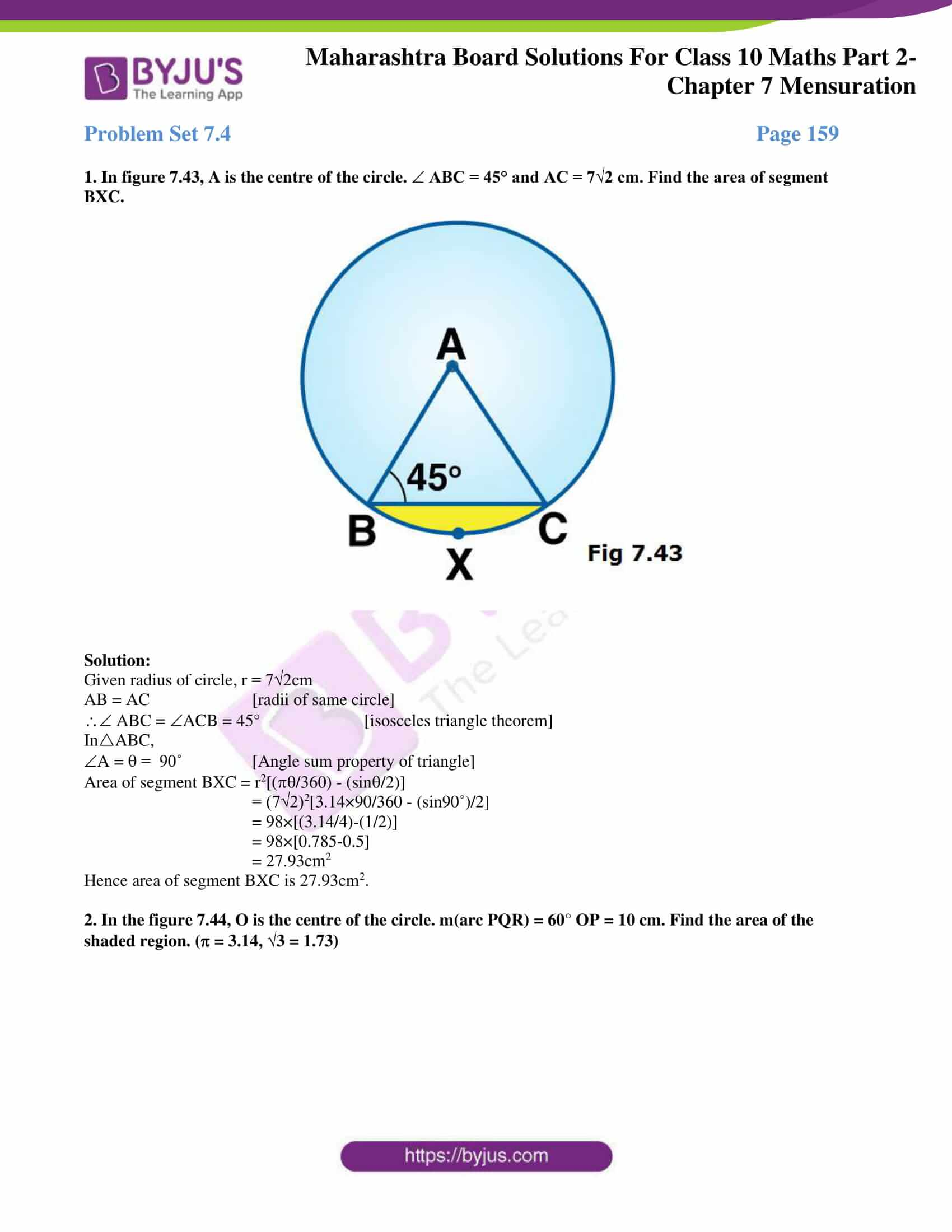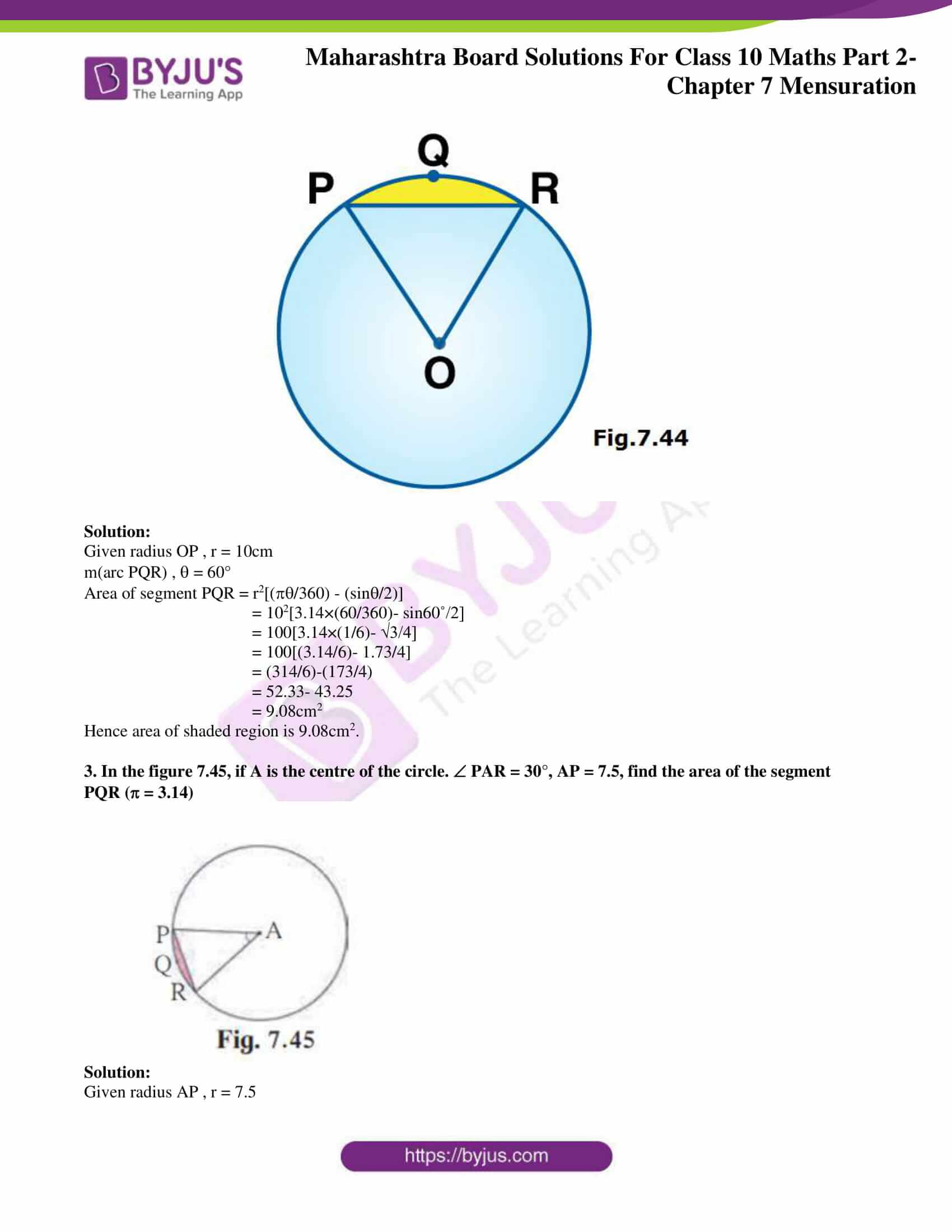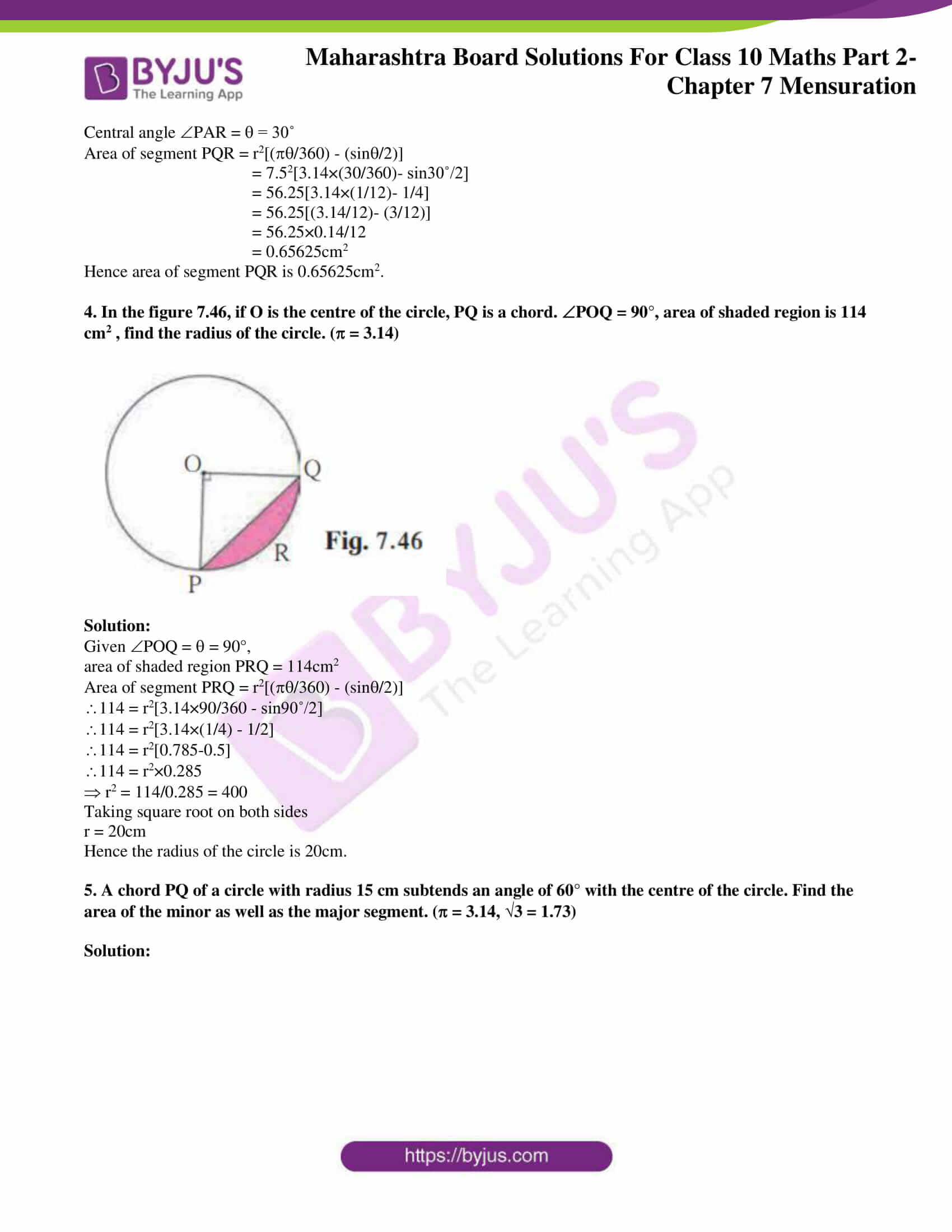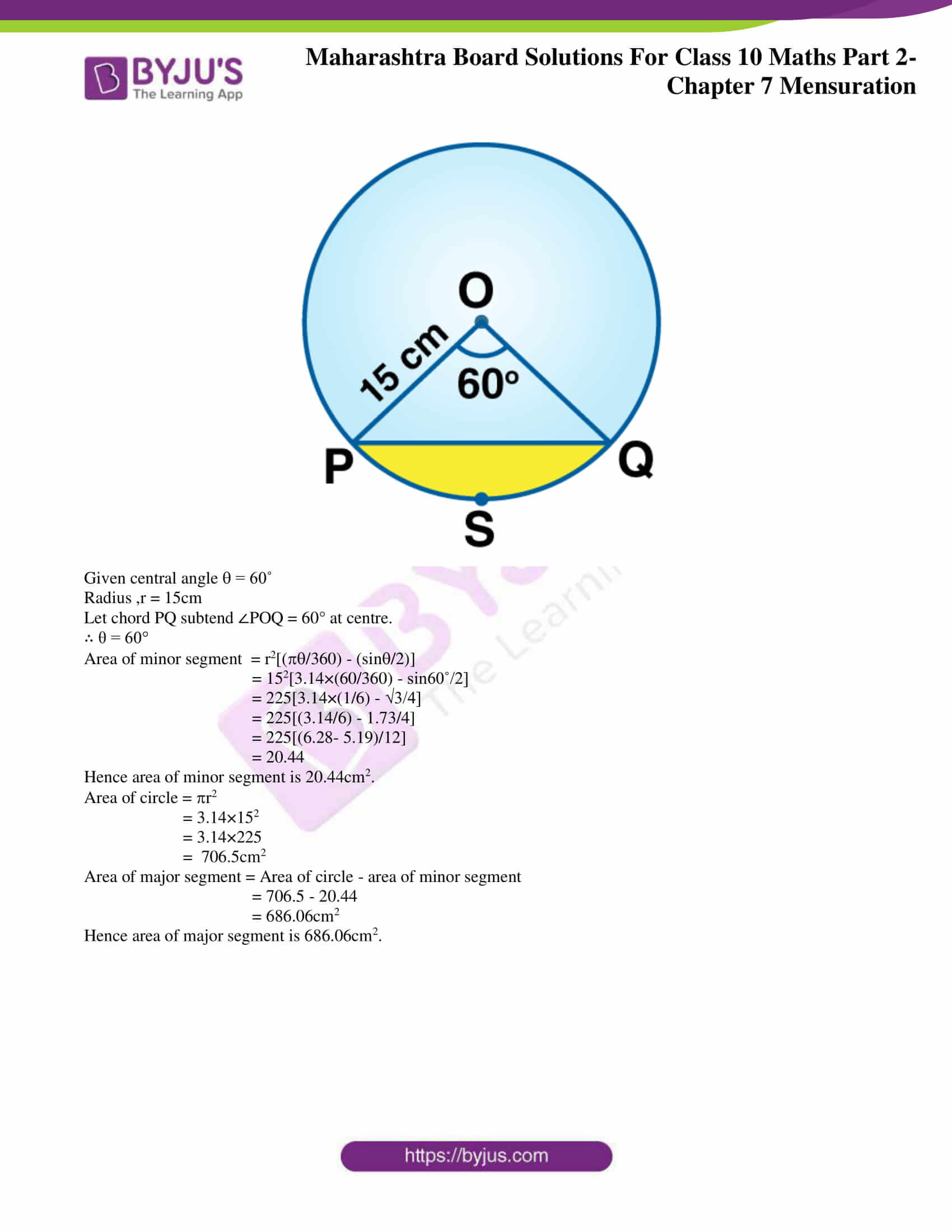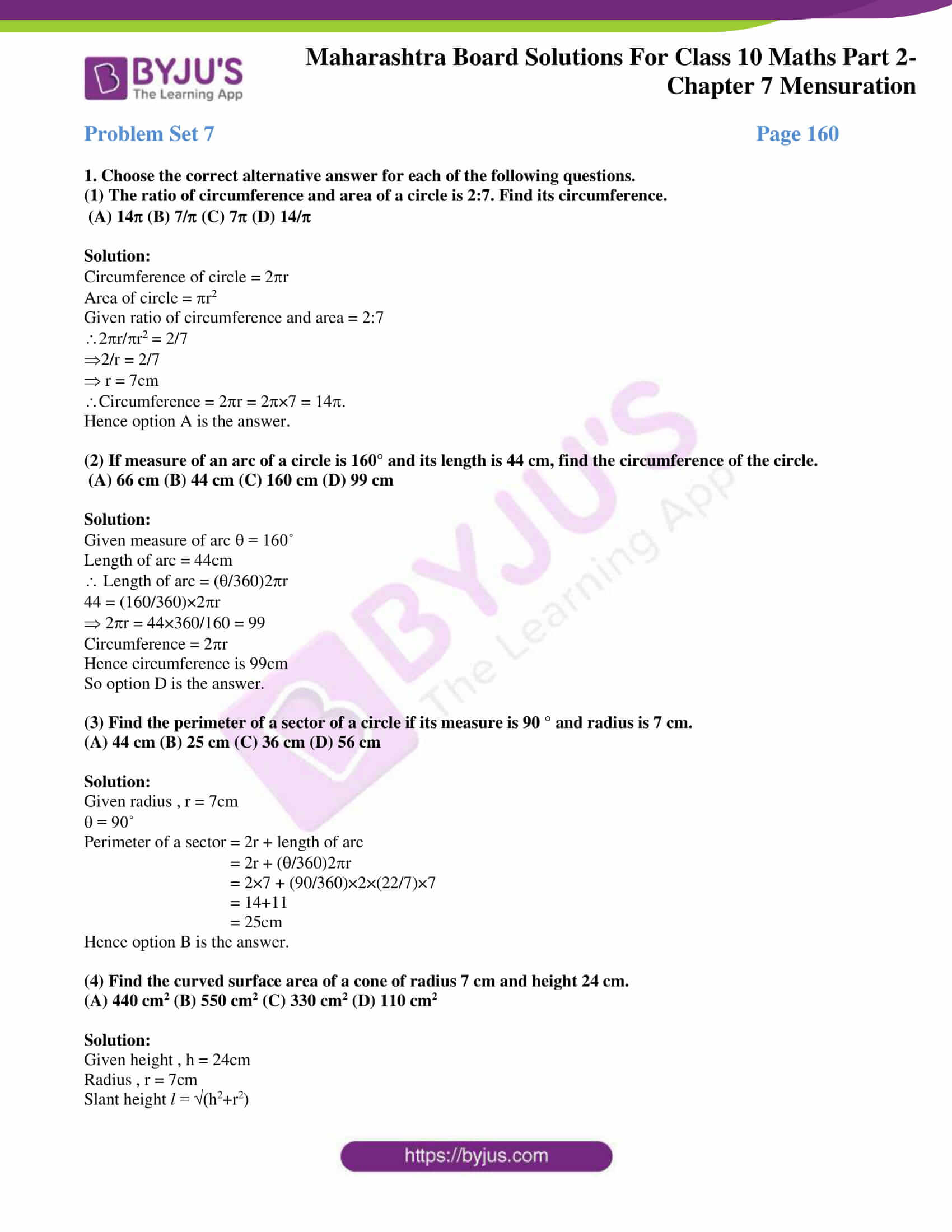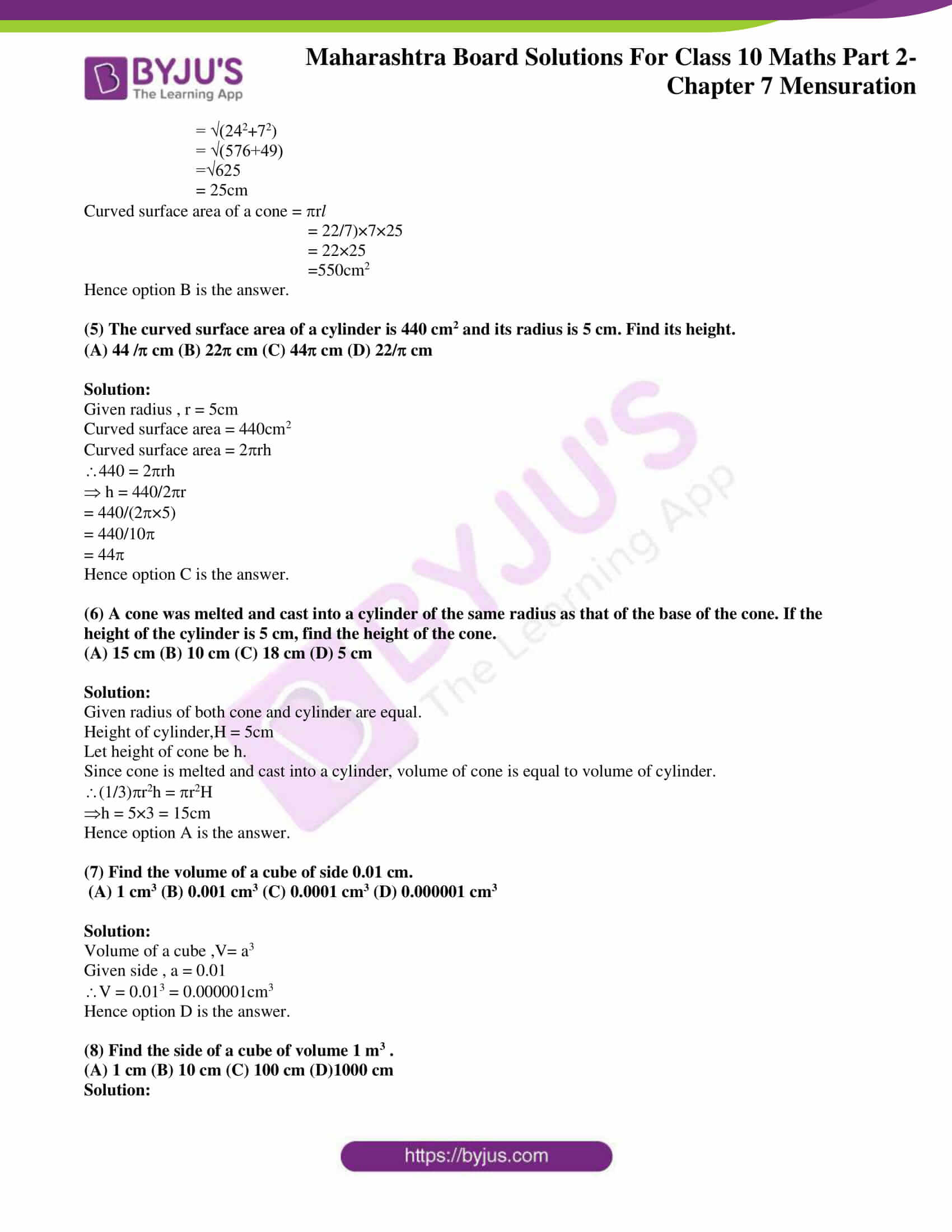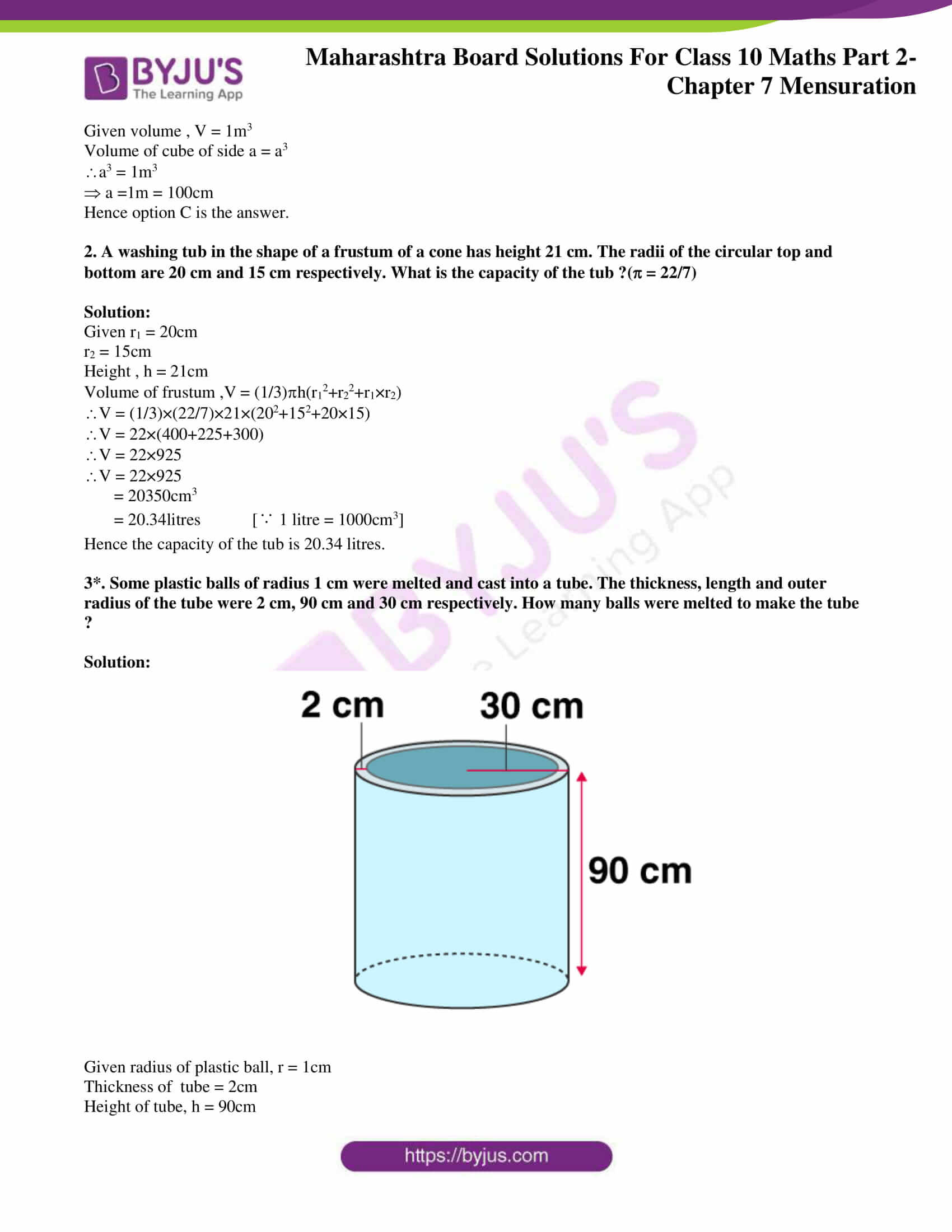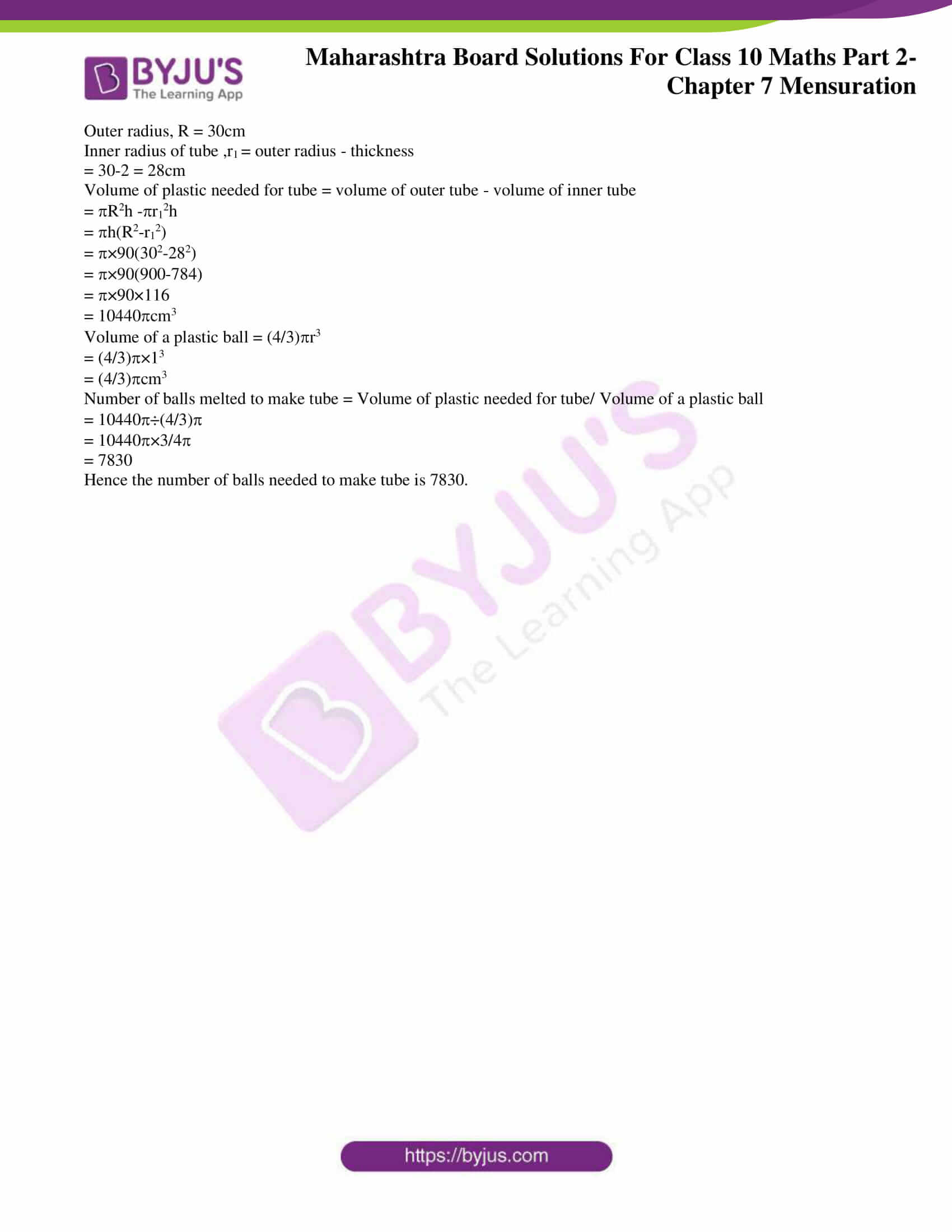### Access answers to Maths MSBSHSE Solutions For SSC Part 2 Chapter 7 – Mensuration

Practice Set 7.1 Page 145

1. Find the volume of a cone if the radius of its base is 1.5 cm and its perpendicular height is 5 cm.

Solution:

Given radius of cone ,r = 1.5cm

Height of cone ,h = 5cm

Volume of the cone , V = (1/3)r2h

V = (1/3)×(22/7) ×1.52×5

V = 11.785 cm3

= 11.79 cm3

Hence the volume of the cone is 11.79 cm3.

2. Find the volume of a sphere of diameter 6 cm.

Solution:

Given diameter of sphere , d = 6cm

Radius r = d/2 = 3

Volume of a sphere, V = (4/3)r3

V = (4/3)×(22/7)×33

V = 113.14cm3

Hence the volume of the sphere is 113.14cm3.

3. Find the total surface area of a cylinder if the radius of its base is 5 cm and height is 40 cm.

Solution:

Given radius of cylinder , r = 5cm

Height of cylinder, h = 40cm

Total surface area of cylinder = 2r(r+h)

= 2×(22/7)×5×(5+40)

= 2×(22/7)×5×45

= 1414.28cm2

Hence Total surface area of cylinder is 1414.28cm2.

4. Find the surface area of a sphere of radius 7 cm.

Solution:

Given radius of sphere , r = 7cm

Surface area, A = 4r2

A = 4×(22/7)×72

= 616 cm2

Hence surface area of sphere is 616cm2.

5. The dimensions of a cuboid are 44 cm, 21 cm, 12 cm. It is melted and a cone of height 24 cm is made. Find the radius of its base.

Solution:

Given length of cuboid , l = 44 cm

Breadth of cuboid ,b = 21 cm

Height of cuboid , h = 12cm

Volume of the cuboid , V = lbh

V = 44×21×12 = 11088cm3

Given Height of cone ,h = 24cm

Since cuboid is melted and a cone is made, the volume will be same.

Volume of cone = Volume of cuboid.

(1/3)r2h = 11088

(1/3)×(22/7)×r2×24 = 11088

r2 = 11088/8×(22/7)

r2 = 441

Taking square root

r = 21

Hence radius of the cone is 21 cm.

6. Observe the measures of pots in figure 7.8 and 7.9. How many jugs of water can the cylindrical pot hold ?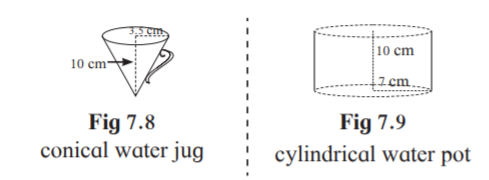Solution:

Given radius of jug , r = 3.5 cm

Height of jug , h = 10 cm

Volume of conical jug, V = (1/3)r2h

= (1/3)××3.52×10 = (122.5/3)×

Given Radius of pot ,R = 7cm

Height of pot, H = 10 cm

Volume of pot = R2H

= ×72×10 = 490

Number of jugs of water the cylindrical pot can hold = Volume of pot/Volume of conical jug

= 490 ÷ (122.5/3)×

= 490×(3/122.5)

= 12

Hence the pot can hold 12 jugs of water.

7. A cylinder and a cone have equal bases. The height of the cylinder is 3 cm and the area of its base is 100 cm2 .The cone is placed upon the cylinder. Volume of the solid figure so formed is 500 cm3 . Find the total height of the figure.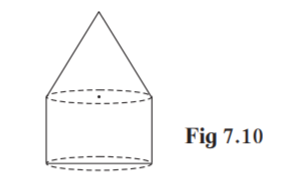Solution:

Given height of cylinder , h = 3cm

Base area of cylinder , r2 = 100cm2 …………..(i)

Volume of solid = 500cm3

Given cylinder and cone have equal bases. the radii will be equal.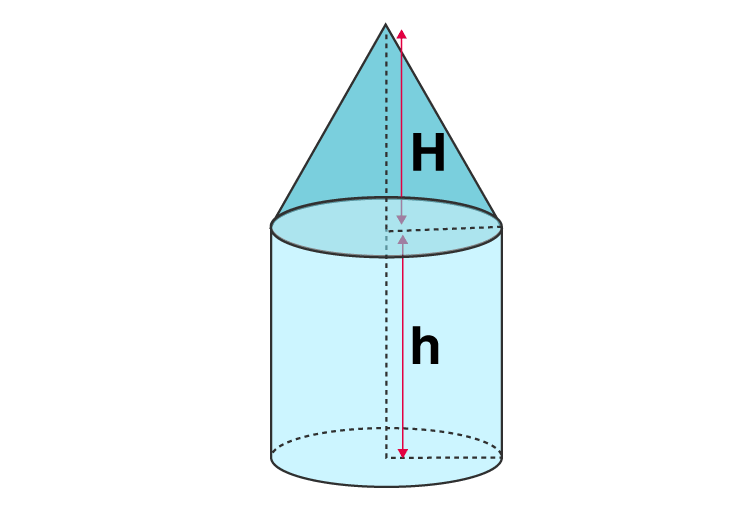Let height of cone be H

Volume of solid = volume of cylinder + volume of cone

500 = r2h+(1/3) r2H

500 = 100×3+(1/3)×100×H [∵r2 = 100, base area of cylinder and cone are equal]

500 = 300+(100/3)H

(100/3)H = 200

H = 3×200/100 = 6

Hence height of cone is 6cm.

Total height of figure = h+H

= 3+6 = 9cm

Hence the total height of the figure is 9cm.

8. In figure 7.11, a toy made from a hemisphere, a cylinder and a cone is shown. Find the total area of the toy.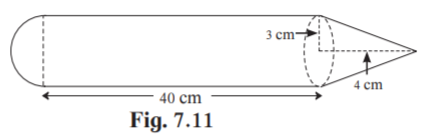Solution:

Given: For the conical Part,

height ,h = 4 cm, radius , r = 3 cm

For the cylindrical part,

height ,H = 40 cm, radius ,r = 3 cm

For the hemispherical part,

Slant height of cone (l) = √(h2+r2)

= √(42+32) = √(16+9)

= √25 = 5 cm

Curved surface area of hemisphere = 2πr2

= 2×π×32

= 18π cm2

Curved surface area of cylinder = 2πrH

= 2×π×3×40

= 240π cm2

Curved surface area of cone = πrl

= π×3×5

= 15π cm2

Total area of the toy

= Curved surface area of cone + Curved surface area of cylinder + Curved surface area of hemisphere

= 15π + 240π + 18π

= 273 π cm2

Hence total area of the toy is 273π cm2.

9. In the figure 7.12, a cylindrical wrapper of flat tablets is shown. The radius of a tablet is 7 mm and its thickness is 5 mm. How many such tablets are wrapped in the wrapper ?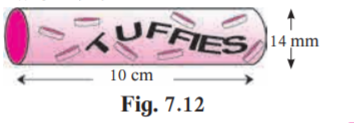Solution:

Given radius of tablet, r = 7mm

Thickness of tablet , h = 5mm

Volume of tablet =

Radius of cylindrical wrapper , R = 14/2 = 7mm

Height of cylindrical wrapper , H = 10cm = 10×0 = 100mm

Let n be the number of tablets that can be wrapped.

n = volume of cylindrical wrapper/volume of tablet

= R2H/r2h

=× 72×100/×72×5

= 20

Hence number of tablets that can be wrapped in wrapper is 20.

10. Figure 7.13 shows a toy. Its lower part is a hemisphere and the upper part is a cone. Find the volume and the surface area of the toy from the measures shown in the figure.(=3.14)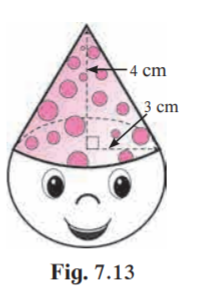Solution:

Given radius of cone ,r = 3 cm

Height of cone ,h = 4 cm

Radius of hemisphere, r = 3cm

Slant height of cone , l = √(h2+r2)

l = √(42+32) = √(16+9) = √25 = 5

Curved surface area of cone = rl

= ×3×5 = 15cm2

Curved surface area of hemisphere = (2/3)r3

= (2/3)×33 = 18cm2

Surface area of toy = Curved surface area of cone+ Curved surface area of hemisphere

= 15+18 = 33 = 33×3.14 = 103.62cm2

Volume of cone = (1/3)r2h

= (1/3)×32×4 = 12cm3

Volume of hemisphere = (2/3)r3

= (2/3)×33 = 18cm3

Volume of toy = Volume of cone+ Volume of hemisphere

= 12+18 = 30 = 30×3.14 = 94.2cm3

Hence the surface area and volume of the toy are 103.62cm2 and 94.2cm3 respectively.

11. Find the surface area and the volume of a beach ball shown in the figure.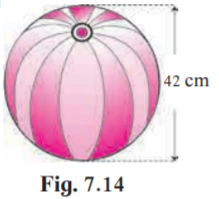Solution:

Given diameter of ball , d = 42 cm

radius of ball , r = 42/2 = 21cm

Surface area of sphere = 4r2

= 4××212 = 4×3.14×441 = 5538.96 cm2

Volume of sphere = (4/3)r3

= (4/3)××213 = (4/3)×3.14×9261 = 38772.72 cm3

Hence the surface area and volume of ball are 5538.96 cm2 and 38772.72 cm3 respectively.

12. As shown in the figure, a cylindrical glass contains water. A metal sphere of diameter 2 cm is immersed in it. Find the volume of the water.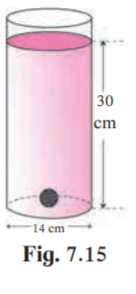Solution:

Given diameter of cylindrical glass , d = 14cm

Radius , R = 14/2 = 7cm

Height of glass in cylindrical glass, H = 30cm

Given diameter of metal sphere = 2cm

radius of metal sphere , r = 2/2 = 1cm

Volume of sphere = (4/3)r3

= (4/3)××13

= (4/3)

= (4/3)×(22/7)

= 4.19cm3

Volume of water with sphere in it = R2H

= ×72×30

= 1470

= 4620cm3

Volume of water in glass = Volume of water with sphere in it – Volume of sphere

= 4620-4.19 = 4615.81cm3

Hence the volume of water in glass is 4615.81cm3.

Practice Set 7.2 Page 148

1. The radii of two circular ends of frustum shape bucket are 14 cm and 7 cm. Height of the bucket is 30 cm. How many liters of water it can hold ? (1 litre = 1000 cm3 )

Solution: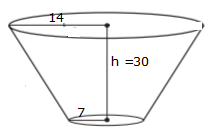Given height of bucket , h = 30cm

r1 = 14cm

r2= 7cm

Volume of a frustum = (1/3)h(r12+r22+r1×r2)

Volume of bucket = (1/3)×30(142+72+14×7)

= 10×(196+49+98)

= 3430

= 10780cm3

= 10.78 litres [∵1 litre = 1000 cm3 ]

Hence the bucket can hold 10.78 litres of water.

2. The radii of ends of a frustum are 14 cm and 6 cm respectively and its height is 6 cm. Find its

i) curved surface area ii) total surface area. iii ) volume ( = 3.14)

Solution:

Given r1 = 14cm

r2= 6cm

Height , h = 6cm

Slant height of frustum l = √[h2+(r1-r2)2]

= √[62+(14-6)2]

= √[36+(8)2]

= √[36+64]

= √100 = 10

(i) Curved surface area of frustum = l(r1+r2)

= ×10(14+6)

= ×10×20

= 3.14 ×200

= 628cm2

Hence curved surface area of frustum is 628cm2.

(ii) Total surface area of frustum = l (r1+ r2)+r12+r22

= ×10(14+ 6)+×142+×62

= ×10×20+×196+×36

= 200+196+36

= 432

= 432×3.14

= 1356.48cm2

Hence Total surface area of frustum is 1356.48cm2.

(iii)Volume of frustum = (1/3)h(r12+r22+r1×r2)

= (1/3)×6(142+62+14×6)

= 2×(196+36+84)

= 2×3.14×316

= 1984.48cm3

Hence volume of frustum is 1984.48cm3

3. The circumferences of circular faces of a frustum are 132 cm and 88 cm and its height is 24 cm. To find the curved surface area of the frustum complete the following activity. ( = 22/7 ).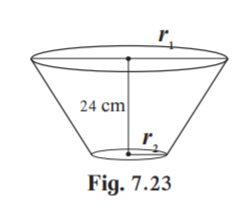Circumference1 = 2r1 = 132

r1 = 132/2 = ____

Circumference2 = 2r2 = 88

r2 = 88/2 = ____

Slant height of frustum l = √[h2+(r1-r2)2]

= √[__2+(___)2]

= ____cm

Curved surface area of frustum = (r1+r2)l

= ×___×___

= ___sq.cm.

Solution:

Circumference1 = 2r1 = 132

r1 = 132/2 = 21cm [=22/7]

Circumference2 = 2r2 = 88

r2 = 88/2 = 14cm

Slant height of frustum l = √[h2+(r1-r2)2]

= √[242+(21-14)2] [given h =24]

= √[576+(7)2]

= √[576+49]

= √625

= 25cm

Curved surface area of frustum = (r1+r2)l

= ×(21+14)×25

= ×(35)×25

= 2750sq.cm

Practice set 7.3 Page 154

1. Radius of a circle is 10 cm. Measure of an arc of the circle is 54°. Find the area of the sector associated with the arc. ( = 3.14 )

Solution:

Given radius of circle , r = 10cm

Measure of an arc of the circle , = 54˚

= 3.14

Area of sector , A = (/360)r2

A = (54/360)×3.14×102

= (9/60)×3.14×100

= 47.1cm2

Hence area of the sector is 47.1cm2

2. Measure of an arc of a circle is 80˚ and its radius is 18 cm. Find the length of the arc. ( = 3.14)

Solution:

Given measure of arc, = 80˚

= 3.14

Length of arc , l = (/360)2r

l = (80/360)×2×3.14×18

= 25.12cm

Hence the length of the arc is 25.12cm.

3. Radius of a sector of a circle is 3.5 cm and length of its arc is 2.2 cm. Find the area of the sector.

Solution:

Given radius of sector of a circle , r = 3.5cm

Length of the arc, l = 2.2cm

Area of sector ,A = lr/2

A = 2.2×3.5/2 = 3.85cm2

Hence the area of the sector is 3.85cm2

4. Radius of a circle is 10 cm. Area of a sector of the sector is 100 cm2 . Find the area of its corresponding major sector. ( = 3.14 )

Solution:

Given radius of circle , r = 10cm

Area of minor sector , A = 100cm2

Area of circle = r2 = 3.14×102 = 3.14×100 = 314cm2

Area of major sector = Area of circle – Area of minor sector

= 314-100

= 214cm2

Hence the area of the major sector is 214cm2

5. Area of a sector of a circle of radius 15 cm is 30 cm2 . Find the length of the arc of the sector.

Solution:

Given radius of circle , r = 15cm

Area of the sector of circle , A = 30cm2

Area of sector = length of arc ×radius/2 = lr/2

Length of arc , l = 2A/r = 2×30/15 = 4cm

Hence the length of the arc is 4cm.

6. In the figure 7.31, radius of the circle is 7 cm and m(arc MBN) = 60°, find

(1) Area of the circle .

(2) A(O – MBN) .

(3) A(O – MCN) .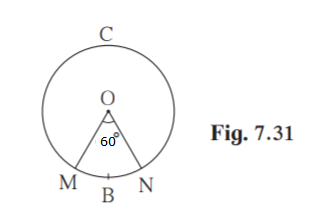Solution:

Given radius of circle , r = 7cm

(1) Area of the circle , A = r2

= (22/7)×72

=( 22/7)×49

= 154cm2

Hence the area of the circle is 154cm2

(2)Given m(arc MBN), = 60°

Area of sector = (/360)r2

A(O-MBN) = (60/360)×(22/7)×72

= (1/6)×22×7

= 25.67cm2

Hence area of sector O-MBN is 25.67cm2

(3) Area of major sector = Area of circle -Area of minor sector

A(O-MCN) = 154-25.67

= 128.33cm2

Hence area of sector O-MCN is 128.33cm2

7. In figure 7.32, radius of circle is 3.4 cm and perimeter of sector P-ABC is 12.8 cm. Find A(P-ABC).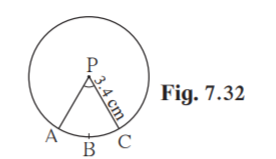Solution:

Given radius , r = 3.4cm

Perimeter of sector P-ABC = PA+arc ABC +PC

12.8 = 3.4+ length of arc ABC +3.4

length of arc ABC , l = 12.8-3.4-3.4 = 6cm

Area of sector P-ABC = lr/2

= 6×3.4/2

= 3×3.4

= 10.2cm2

Hence A(P-ABC) is 10.2cm2.

8. In figure 7.33 O is the centre of the sector. ROQ = MON = 60°. OR = 7 cm, and OM = 21 cm. Find the lengths of arc RXQ and arc MYN. ( = 22/7 )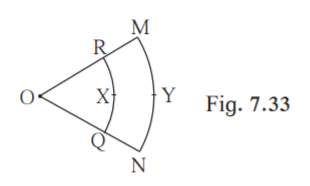Solution:

Given ROQ = MON ,= 60°.

Radius OR, r = 7 cm

Radius OM, R = 21 cm

Length of arc RXQ = (/360)2r

= (60/360)×2×(22/7)×7

= (1/6)× 2×22

= 22/3

= 7.33cm

Hence Length of arc RXQ is 7.33cm.

Length of arc MYN = (/360)2R

= (60/360)×2×(22/7)×21

= (1/6)× 2×22×3

= 22cm

Hence Length of arc MYN is 22cm.

9. In figure 7.34, if A(P-ABC) = 154 cm2 radius of the circle is 14 cm,

find (1) APC.

(2) l(arc ABC) .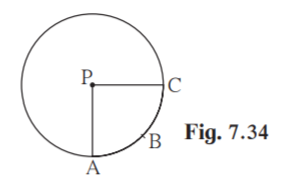Solution:

Given radius of circle , r = 14cm

Area ,A(P-ABC) = 154cm2

(1) Let APC =

A(P-ABC) = (/360)r2

154 = (/360)×(22/7)×142

= (154×360×7)/(22×14×14)

= 90˚

Hence APC is 90˚.

(2)length of arc, l(arc ABC) = (/360)2r

l(arc ABC) = (90/360)×2×(22/7)×14

= (1/4)×2×22×2

= 22cm.

Hence l(arc ABC) is 22cm.

10. Radius of a sector of a circle is 7 cm. If measure of arc of the sector is –

(1) 30°

(2) 210°

(3 ) three right angles;

find the area of the sector in each case.

Solution:

Given radius of sector, r = 7cm

(1)Measure of arc, = 30˚

Area of sector = (/360)r2

= (30/360)×(22/7)×72

= (1/12)×(22/7)×49

= (1/12)×22×7

= 12.83cm2

Hence the area of sector is 12.83cm2

(2)Measure of arc, = 210˚

Area of sector = (/360)r2

= (210/360)×(22/7)×72

= (7/12)×(22/7)×49

= (7/12)×22×7

= 89.83cm2

Hence the area of sector is 89.83cm2

(3)Measure of arc, = 3 right angles = 3×90 = 270˚

Area of sector = (/360)r2

= (270/360)×(22/7)×72

= (3/4)×(22/7)×49

= (3/4)×22×7

= 115.5 cm2

Hence the area of sector is 115.5 cm2

11. The area of a minor sector of a circle is 3.85 cm2 and the measure of its central angle is 36°. Find the radius of the circle.

Solution:

Given area of minor sector = 3.85cm2

Measure of central angle, = 36˚

Area of sector = (/360)r2

3.85 = (36/360)×(22/7)×r2

r2 = (3.85×360×7)/(36×22)

r2 = (3.85×10×7)/22

r2 = 12.25

r = 3.5cm

Hence the radius of the circle is 3.5cm

12. In figure 7.35, PQRS is a rectangle. If PQ = 14 cm, QR = 21 cm, find the areas of the parts x, y and z .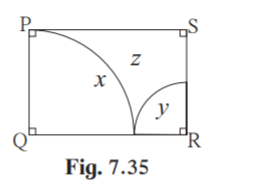Solution: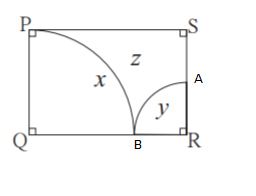Given PQ = 14cm

QR = 21cm

Q = = 90˚

Area of part x = (/360)r2

Area of part x = (90/360)×(22/7)×142

= 11×14

= 154cm2

Consider sector (R-BYA)

QR = QB+BR

BR = 21-14 = 7cm

AR = 7cm [radius of same circle]

Area of part y = (/360)r2

Area of part y = (90/360)×(22/7)×72

= 11×7/2

= 38.5cm2

Area of rectangle PQRS = length ×breadth

= QR×PQ

= 21×14

= 294cm2

Area of part z = Area of rectangle PQRS – [Area of part x+ Area of part y]

Area of part z = 294-(154+38.5)

= 294-192.5

= 101.5cm2

Hence area of part x is 154cm2 , area of part y is 38.5cm2 and area of part z is 101.5cm2

13. LMN is an equilateral triangle. LM = 14 cm. As shown in figure, three sectors are drawn with vertices as centres and radius7 cm. Find,

(1) A ( LMN)

(2) Area of any one of the sectors.

(3) Total area of all the three sectors.

(4) Area of the shaded region.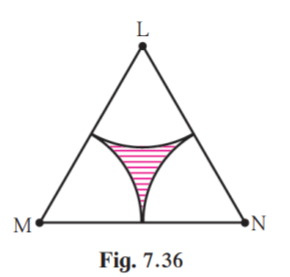Solution:

(1) Given LMN is an equilateral triangle .

LM = 14 cm

Area of LMN = (√3/4)a2

Here a represents the side of equilateral triangle.

a = 14

Area of LMN = (√3/4)×142

= 49√3

= 84.87cm2

Hence area of LMN is 84.87cm2

(2) Since LMN is equilateral, L = M = N = 60˚

= 60˚

Given r = 7

Area of sector = (/360)r2

Area of sector = (60/360)×22/7×72

= 11×7/3

= 25.67cm2

Hence area of one sector = 25.67cm2

(3)Total area of 3 sectors = 3×area of one sector

= 3×25.67

= 77.01cm2

Hence total area of 3 sectors is 77.01cm2.

(4)Area of shaded region = Area of LMN – Area of 3 sectors

= 84.87 – 77.01

= 7.86cm2

Hence area of shaded region is 7.86cm2.

Problem Set 7.4 Page 159

1. In figure 7.43, A is the centre of the circle. ABC = 45° and AC = 7√2 cm. Find the area of segment BXC.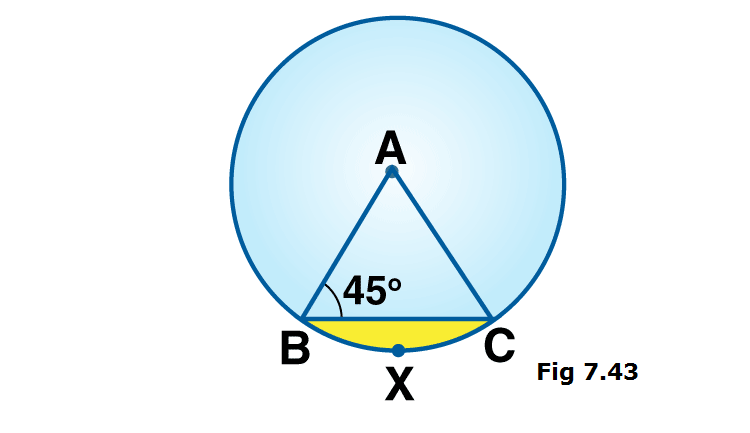Solution:

Given radius of circle, r = 7√2cm

AB = AC [radii of same circle]

ABC = ACB = 45° [isosceles triangle theorem]

InABC,

A = = 90˚ [Angle sum property of triangle]

Area of segment BXC = r2[(/360) – (sin/2)]

= (7√2)2[3.14×90/360 – (sin90˚)/2]

= 98×[(3.14/4)-(1/2)]

= 98×[0.785-0.5]

= 27.93cm2

Hence area of segment BXC is 27.93cm2.

2. In the figure 7.44, O is the centre of the circle. m(arc PQR) = 60° OP = 10 cm. Find the area of the shaded region. ( = 3.14, √3 = 1.73)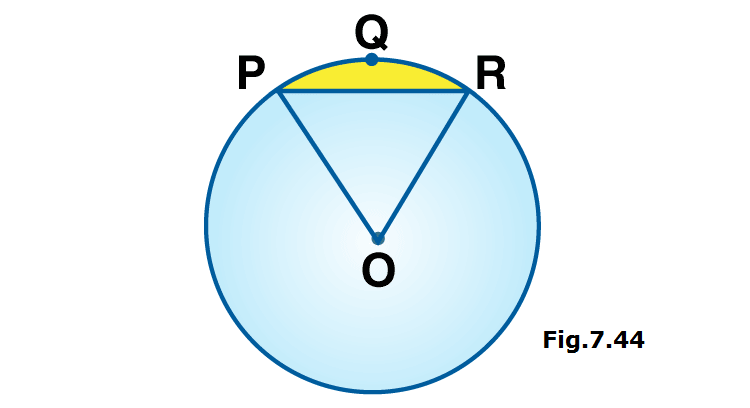Solution:

Given radius OP , r = 10cm

m(arc PQR) , = 60°

Area of segment PQR = r2[(/360) – (sin/2)]

= 102[3.14×(60/360)- sin60˚/2]

= 100[3.14×(1/6)- √3/4]

= 100[(3.14/6)- 1.73/4]

= (314/6)-(173/4)

= 52.33- 43.25

= 9.08cm2

Hence area of shaded region is 9.08cm2.

3. In the figure 7.45, if A is the centre of the circle. PAR = 30°, AP = 7.5, find the area of the segment PQR ( = 3.14)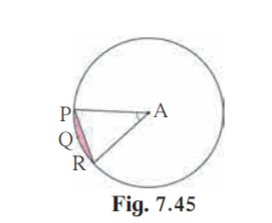Solution:

Given radius AP , r = 7.5

Central angle PAR = = 30˚

Area of segment PQR = r2[(/360) – (sin/2)]

= 7.52[3.14×(30/360)- sin30˚/2]

= 56.25[3.14×(1/12)- 1/4]

= 56.25[(3.14/12)- (3/12)]

= 56.25×0.14/12

= 0.65625cm2

Hence area of segment PQR is 0.65625cm2.

4. In the figure 7.46, if O is the centre of the circle, PQ is a chord. POQ = 90°, area of shaded region is 114 cm2 , find the radius of the circle. ( = 3.14)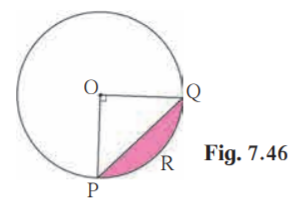Solution:

Given POQ = = 90°,

area of shaded region PRQ = 114cm2

Area of segment PRQ = r2[(/360) – (sin/2)]

114 = r2[3.14×90/360 – sin90˚/2]

114 = r2[3.14×(1/4) – 1/2]

114 = r2[0.785-0.5]

114 = r2×0.285

r2 = 114/0.285 = 400

Taking square root on both sides

r = 20cm

Hence the radius of the circle is 20cm.

5. A chord PQ of a circle with radius 15 cm subtends an angle of 60° with the centre of the circle. Find the area of the minor as well as the major segment. ( = 3.14, √3 = 1.73)

Solution: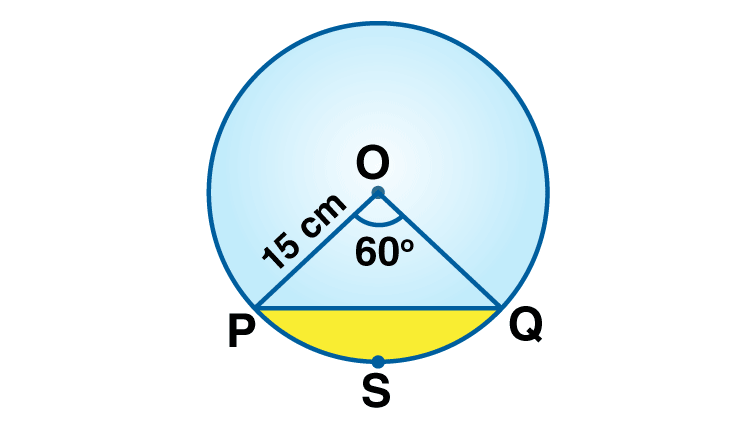Given central angle = 60˚

Let chord PQ subtend ∠POQ = 60° at centre.

∴ θ = 60°

Area of minor segment = r2[(/360) – (sin/2)]

= 152[3.14×(60/360) – sin60˚/2]

= 225[3.14×(1/6) – √3/4]

= 225[(3.14/6) – 1.73/4]

= 225[(6.28- 5.19)/12]

= 20.44

Hence area of minor segment is 20.44cm2.

Area of circle = r2

= 3.14×152

= 3.14×225

= 706.5cm2

Area of major segment = Area of circle – area of minor segment

= 706.5 – 20.44

= 686.06cm2

Hence area of major segment is 686.06cm2.

Problem Set 7 Page 160

1. Choose the correct alternative answer for each of the following questions.

(1) The ratio of circumference and area of a circle is 2:7. Find its circumference.

(A) 14 (B) 7/ (C) 7 (D) 14/

Solution:

Circumference of circle = 2r

Area of circle = r2

Given ratio of circumference and area = 2:7

2r/r2 = 2/7

2/r = 2/7

r = 7cm

Circumference = 2r = 2×7 = 14.

Hence option A is the answer.

(2) If measure of an arc of a circle is 160° and its length is 44 cm, find the circumference of the circle.

(A) 66 cm (B) 44 cm (C) 160 cm (D) 99 cm

Solution:

Given measure of arc = 160˚

Length of arc = 44cm

Length of arc = (/360)2r

44 = (160/360)×2r

2r = 44×360/160 = 99

Circumference = 2r

Hence circumference is 99cm

So option D is the answer.

(3) Find the perimeter of a sector of a circle if its measure is 90 ° and radius is 7 cm.

(A) 44 cm (B) 25 cm (C) 36 cm (D) 56 cm

Solution:

Given radius , r = 7cm

= 90˚

Perimeter of a sector = 2r + length of arc

= 2r + (/360)2r

= 2×7 + (90/360)×2×(22/7)×7

= 14+11

= 25cm

Hence option B is the answer.

(4) Find the curved surface area of a cone of radius 7 cm and height 24 cm.

(A) 440 cm2 (B) 550 cm2 (C) 330 cm2 (D) 110 cm2

Solution:

Given height , h = 24cm

Slant height l = √(h2+r2)

= √(242+72)

= √(576+49)

=√625

= 25cm

Curved surface area of a cone = rl

= 22/7)×7×25

= 22×25

=550cm2

Hence option B is the answer.

(5) The curved surface area of a cylinder is 440 cm2 and its radius is 5 cm. Find its height.

(A) 44 / cm (B) 22 cm (C) 44 cm (D) 22/ cm

Solution:

Given radius , r = 5cm

Curved surface area = 440cm2

Curved surface area = 2rh

440 = 2rh

h = 440/2r

= 440/(2×5)

= 440/10

= 44

Hence option C is the answer.

(6) A cone was melted and cast into a cylinder of the same radius as that of the base of the cone. If the height of the cylinder is 5 cm, find the height of the cone.

(A) 15 cm (B) 10 cm (C) 18 cm (D) 5 cm

Solution:

Given radius of both cone and cylinder are equal.

Height of cylinder,H = 5cm

Let height of cone be h.

Since cone is melted and cast into a cylinder, volume of cone is equal to volume of cylinder.

(1/3)r2h = r2H

h = 5×3 = 15cm

Hence option A is the answer.

(7) Find the volume of a cube of side 0.01 cm.

(A) 1 cm3 (B) 0.001 cm3 (C) 0.0001 cm3 (D) 0.000001 cm3

Solution:

Volume of a cube ,V= a3

Given side , a = 0.01

V = 0.013 = 0.000001cm3

Hence option D is the answer.

(8) Find the side of a cube of volume 1 m3 .

(A) 1 cm (B) 10 cm (C) 100 cm (D)1000 cm

Solution:

Given volume , V = 1m3

Volume of cube of side a = a3

a3 = 1m3

a =1m = 100cm

Hence option C is the answer.

2. A washing tub in the shape of a frustum of a cone has height 21 cm. The radii of the circular top and bottom are 20 cm and 15 cm respectively. What is the capacity of the tub ?( = 22/7)

Solution:

Given r1 = 20cm

r2 = 15cm

Height , h = 21cm

Volume of frustum ,V = (1/3)h(r12+r22+r1×r2)

V = (1/3)×(22/7)×21×(202+152+20×15)

V = 22×(400+225+300)

V = 22×925

V = 22×925

= 20350cm3

= 20.34litres [∵ 1 litre = 1000cm3]

Hence the capacity of the tub is 20.34 litres.

3*. Some plastic balls of radius 1 cm were melted and cast into a tube. The thickness, length and outer radius of the tube were 2 cm, 90 cm and 30 cm respectively. How many balls were melted to make the tube ?

Solution: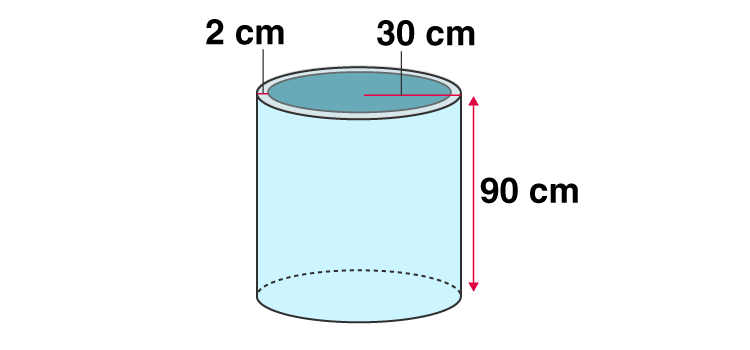Given radius of plastic ball, r = 1cm

Thickness of tube = 2cm

Height of tube, h = 90cm

= 30-2 = 28cm

Volume of plastic needed for tube = volume of outer tube – volume of inner tube

= R2h -r12h

= h(R2-r12)

= ×90(302-282)

= ×90(900-784)

= ×90×116

= 10440cm3

Volume of a plastic ball = (4/3)r3

= (4/3)×13

= (4/3)cm3

Number of balls melted to make tube = Volume of plastic needed for tube/ Volume of a plastic ball

= 10440÷(4/3)

= 10440×3/4

= 7830

Hence the number of balls needed to make tube is 7830.

Mensuration is the part of Geometry, which deals with study of various geometrical shapes, their areas and volumes. In Class 10 Maths Mensuration, we come across volumes and surface areas of three dimensional figures. Log on to BYJU’S to get more information on mathematical concepts and master Mensuration formulas and problems. Enjoy 100% accurate, step by step solutions on BYJU’S.

## Frequently Asked Questions on Maharashtra State Board Solutions for Class 10 Maths Part 2 Chapter 7 Mensuration

### Can these Maharashtra State Board Class 10 Maths Part 2 Chapter 7 Solutions be used to prepare for the exams?

Yes, these Maharashtra State Board Class 10 Maths Part 2 Chapter 7 Solutions can be very helpful. Students are highly recommended to practise these solutions after revising the subjects, as they set the basis for the questions that could get asked often in the board exams. By referring to these solutions after solving the questions will help the students to identify the mistakes and avoid them in the exams.

### What are the concepts covered in these solutions?

This chapter of Maharashtra State Board Class 10 Solutions deals with surface area and volume of three dimensional figures such as cone, cylinder, sphere, hemisphere and frustum of cone. .